Скачать презентацию CHAPTER 11 Output and Costs Business Microeconomics Instructor

Sess 7 Output and Costs.ppt

• Количество слайдов: 74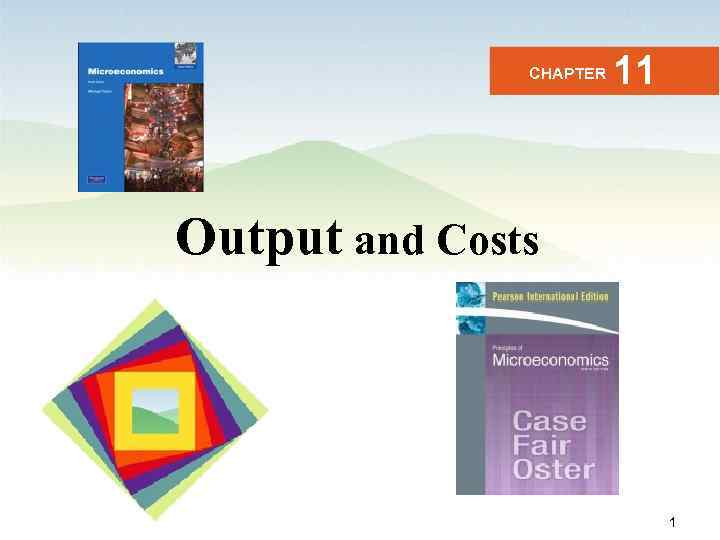CHAPTER 11 Output and Costs Business Microeconomics Instructor: Gulnara Moldasheva 1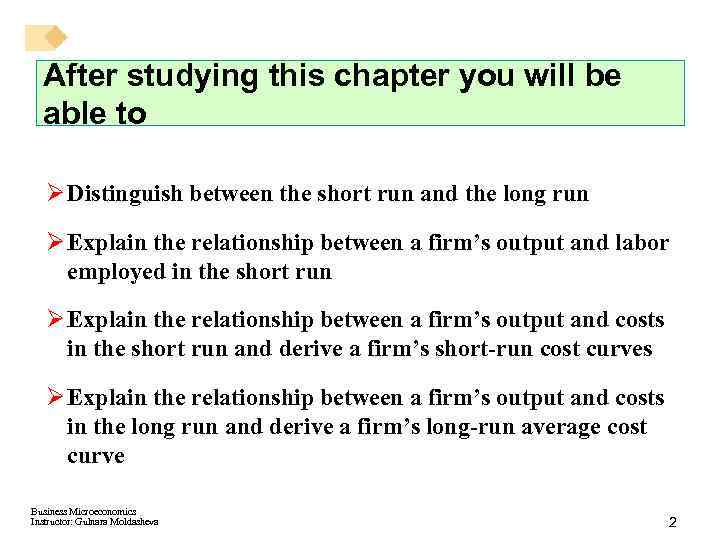After studying this chapter you will be able to Ø Distinguish between the short run and the long run Ø Explain the relationship between a firm’s output and labor employed in the short run Ø Explain the relationship between a firm’s output and costs in the short run and derive a firm’s short-run cost curves Ø Explain the relationship between a firm’s output and costs in the long run and derive a firm’s long-run average cost curve Business Microeconomics Instructor: Gulnara Moldasheva 2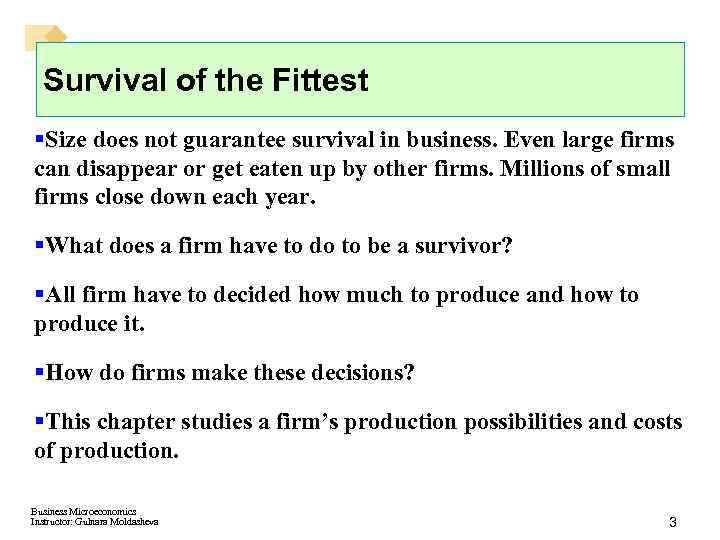Survival of the Fittest §Size does not guarantee survival in business. Even large firms can disappear or get eaten up by other firms. Millions of small firms close down each year. §What does a firm have to do to be a survivor? §All firm have to decided how much to produce and how to produce it. §How do firms make these decisions? §This chapter studies a firm’s production possibilities and costs of production. Business Microeconomics Instructor: Gulnara Moldasheva 3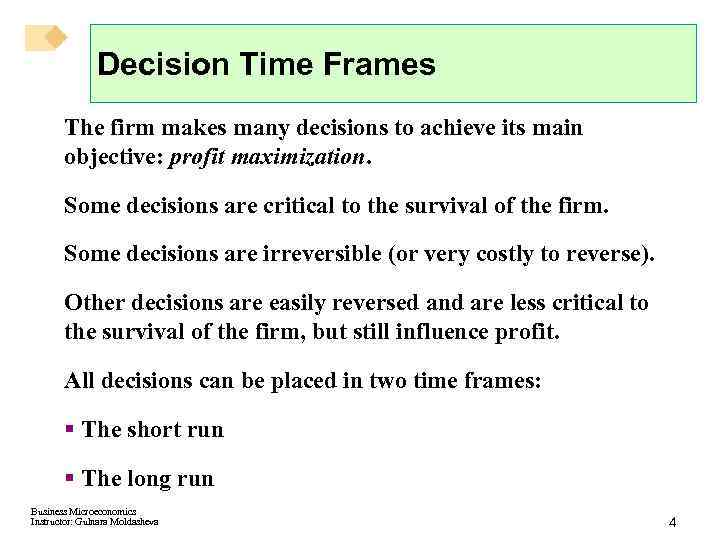Decision Time Frames The firm makes many decisions to achieve its main objective: profit maximization. Some decisions are critical to the survival of the firm. Some decisions are irreversible (or very costly to reverse). Other decisions are easily reversed and are less critical to the survival of the firm, but still influence profit. All decisions can be placed in two time frames: § The short run § The long run Business Microeconomics Instructor: Gulnara Moldasheva 4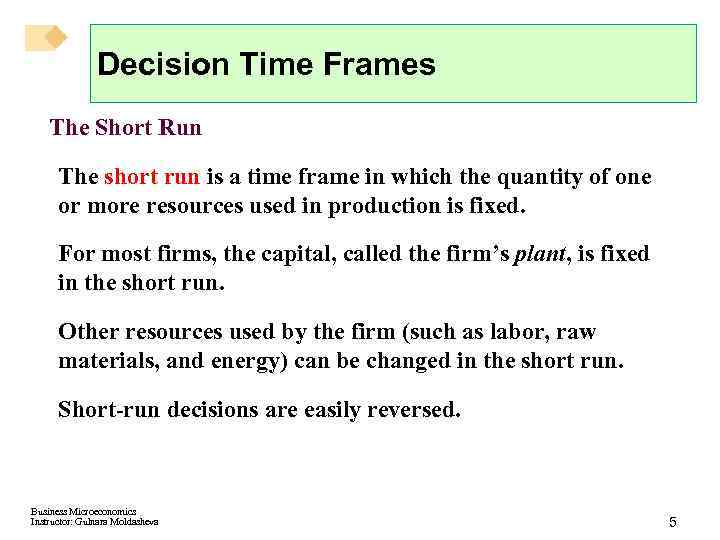Decision Time Frames The Short Run The short run is a time frame in which the quantity of one or more resources used in production is fixed. For most firms, the capital, called the firm’s plant, is fixed in the short run. Other resources used by the firm (such as labor, raw materials, and energy) can be changed in the short run. Short-run decisions are easily reversed. Business Microeconomics Instructor: Gulnara Moldasheva 5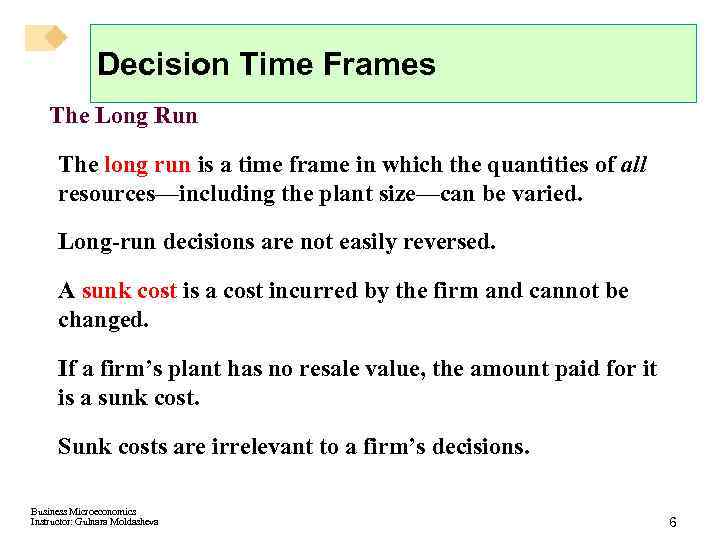Decision Time Frames The Long Run The long run is a time frame in which the quantities of all resources—including the plant size—can be varied. Long-run decisions are not easily reversed. A sunk cost is a cost incurred by the firm and cannot be changed. If a firm’s plant has no resale value, the amount paid for it is a sunk cost. Sunk costs are irrelevant to a firm’s decisions. Business Microeconomics Instructor: Gulnara Moldasheva 6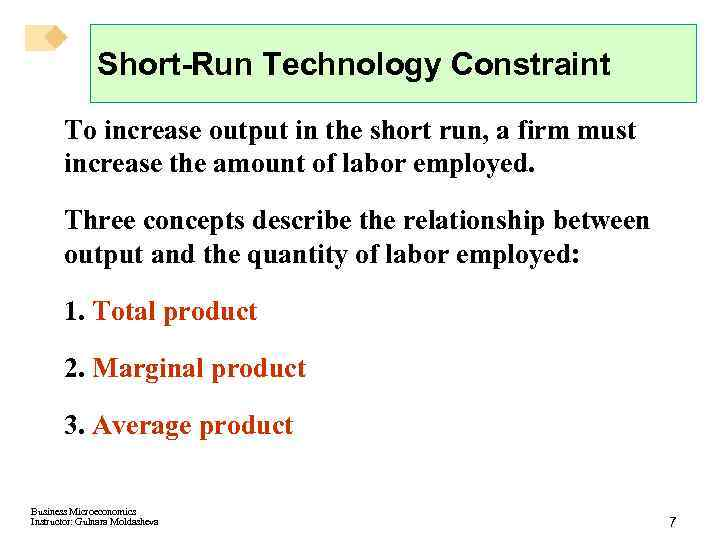Short-Run Technology Constraint To increase output in the short run, a firm must increase the amount of labor employed. Three concepts describe the relationship between output and the quantity of labor employed: 1. Total product 2. Marginal product 3. Average product Business Microeconomics Instructor: Gulnara Moldasheva 7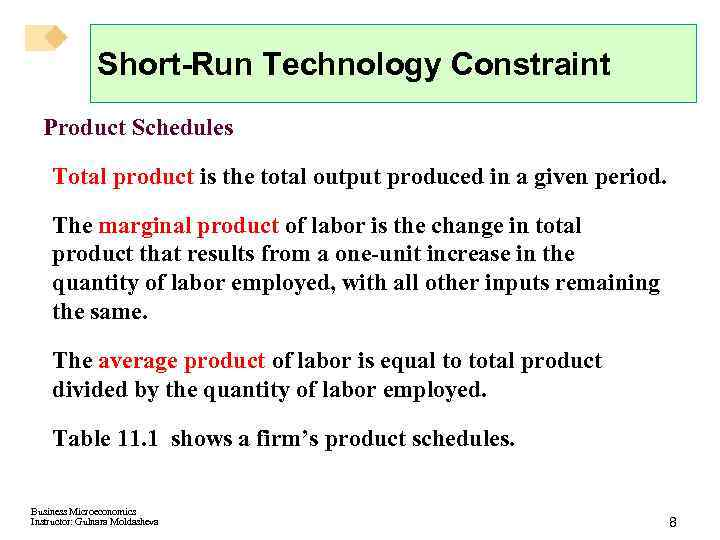Short-Run Technology Constraint Product Schedules Total product is the total output produced in a given period. The marginal product of labor is the change in total product that results from a one-unit increase in the quantity of labor employed, with all other inputs remaining the same. The average product of labor is equal to total product divided by the quantity of labor employed. Table 11. 1 shows a firm’s product schedules. Business Microeconomics Instructor: Gulnara Moldasheva 8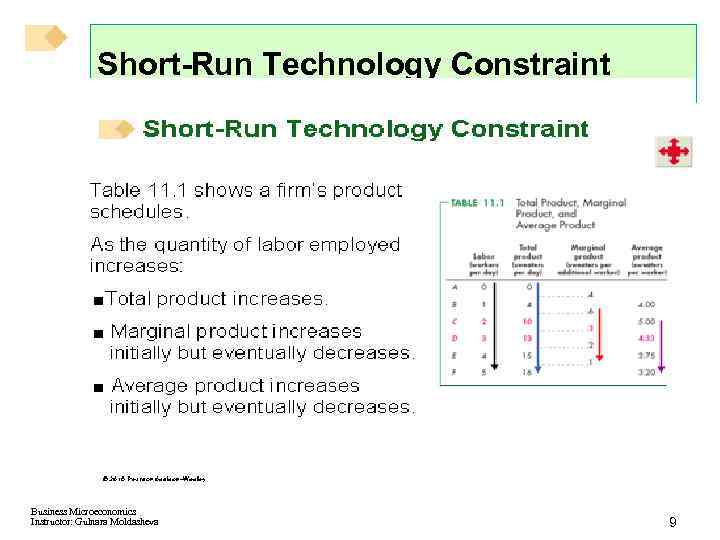Short-Run Technology Constraint Business Microeconomics Instructor: Gulnara Moldasheva 9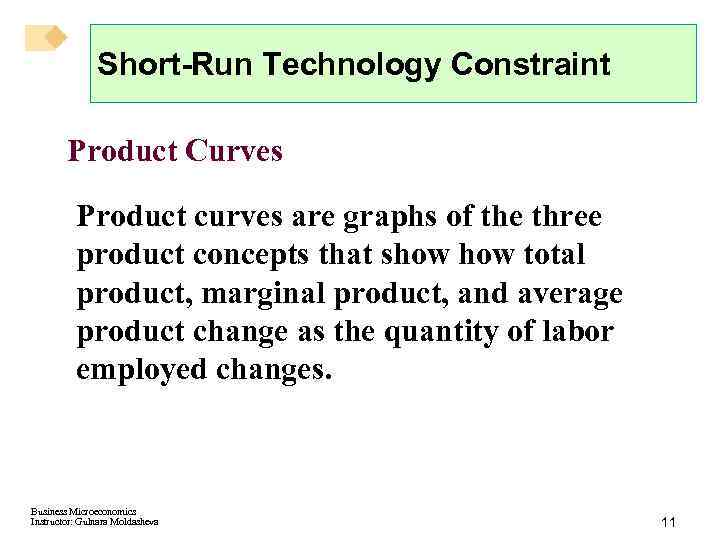Short-Run Technology Constraint Product Curves Product curves are graphs of the three product concepts that show total product, marginal product, and average product change as the quantity of labor employed changes. Business Microeconomics Instructor: Gulnara Moldasheva 11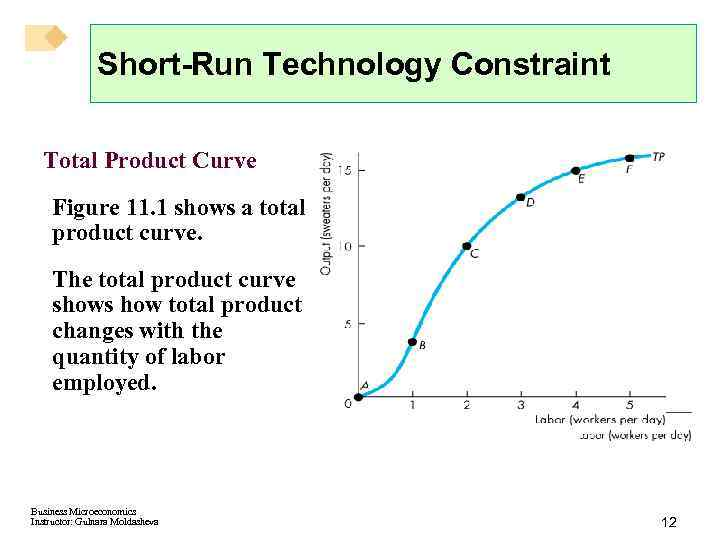Short-Run Technology Constraint Total Product Curve Figure 11. 1 shows a total product curve. The total product curve shows how total product changes with the quantity of labor employed. Business Microeconomics Instructor: Gulnara Moldasheva 12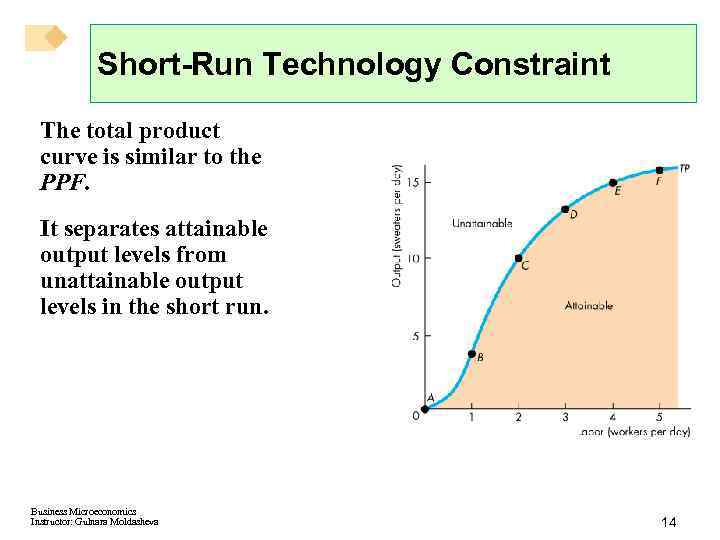Short-Run Technology Constraint The total product curve is similar to the PPF. It separates attainable output levels from unattainable output levels in the short run. Business Microeconomics Instructor: Gulnara Moldasheva 14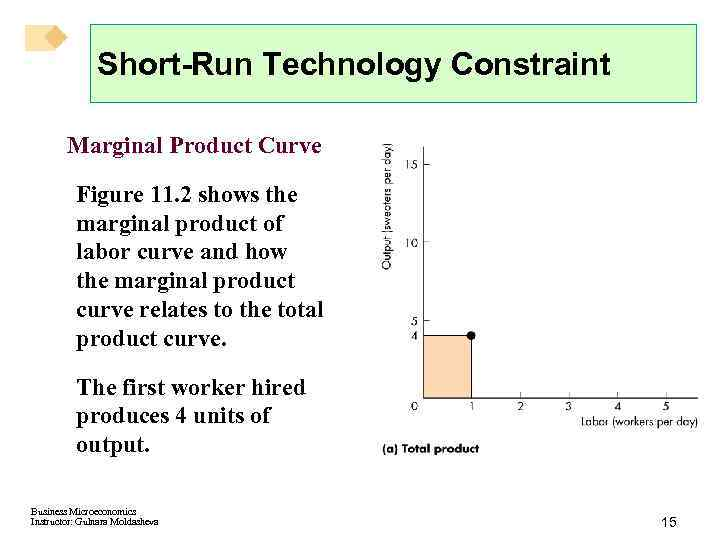Short-Run Technology Constraint Marginal Product Curve Figure 11. 2 shows the marginal product of labor curve and how the marginal product curve relates to the total product curve. The first worker hired produces 4 units of output. Business Microeconomics Instructor: Gulnara Moldasheva 15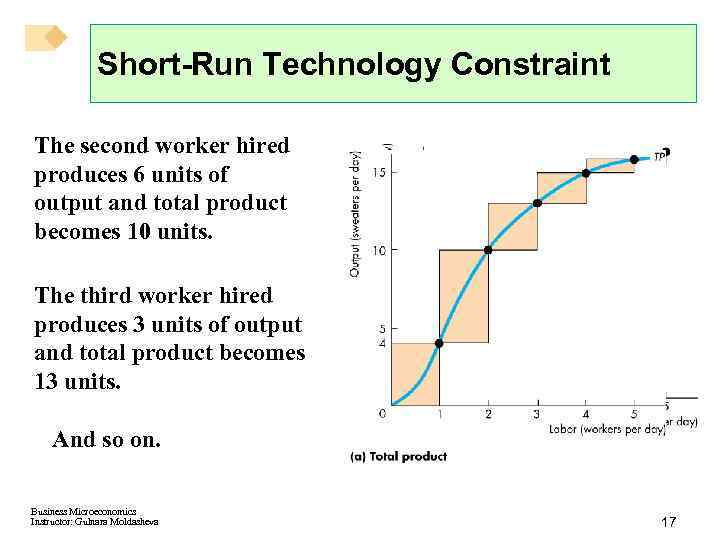Short-Run Technology Constraint The second worker hired produces 6 units of output and total product becomes 10 units. The third worker hired produces 3 units of output and total product becomes 13 units. And so on. Business Microeconomics Instructor: Gulnara Moldasheva 17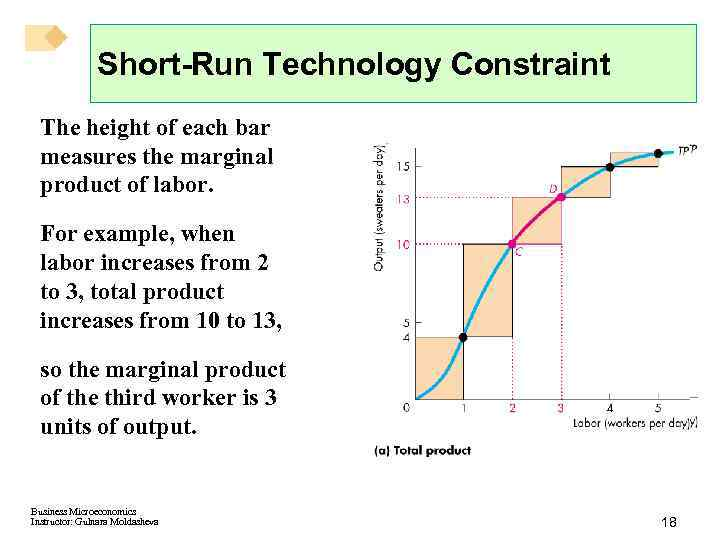Short-Run Technology Constraint The height of each bar measures the marginal product of labor. For example, when labor increases from 2 to 3, total product increases from 10 to 13, so the marginal product of the third worker is 3 units of output. Business Microeconomics Instructor: Gulnara Moldasheva 18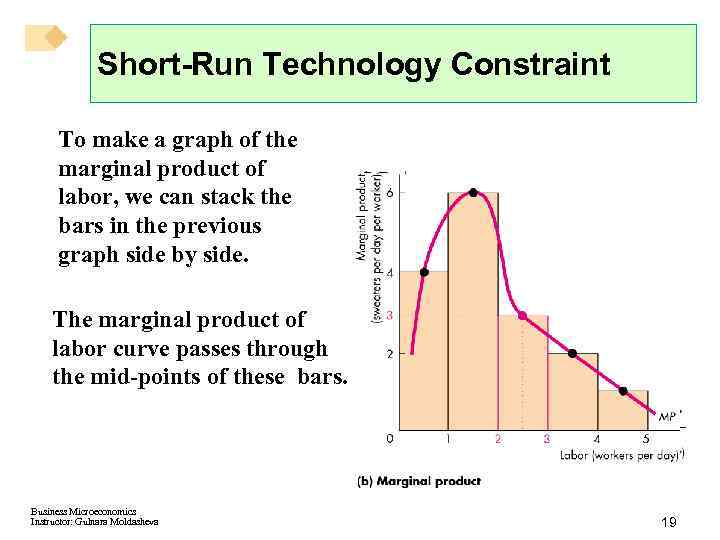Short-Run Technology Constraint To make a graph of the marginal product of labor, we can stack the bars in the previous graph side by side. The marginal product of labor curve passes through the mid-points of these bars. Business Microeconomics Instructor: Gulnara Moldasheva 19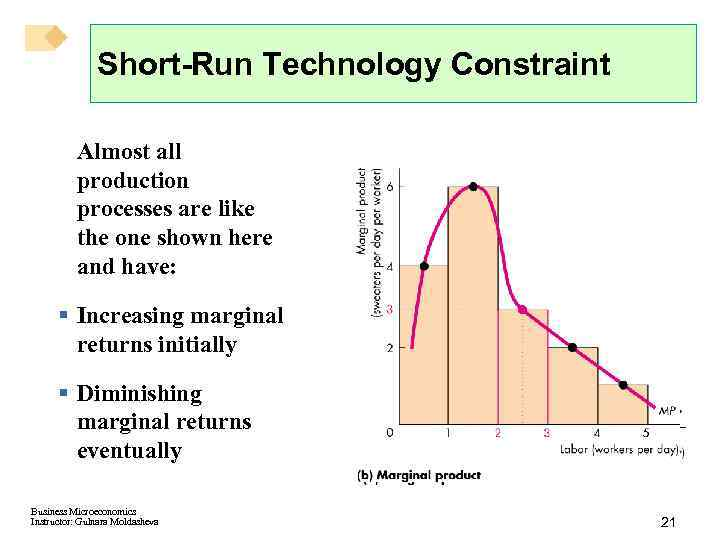Short-Run Technology Constraint Almost all production processes are like the one shown here and have: § Increasing marginal returns initially § Diminishing marginal returns eventually Business Microeconomics Instructor: Gulnara Moldasheva 21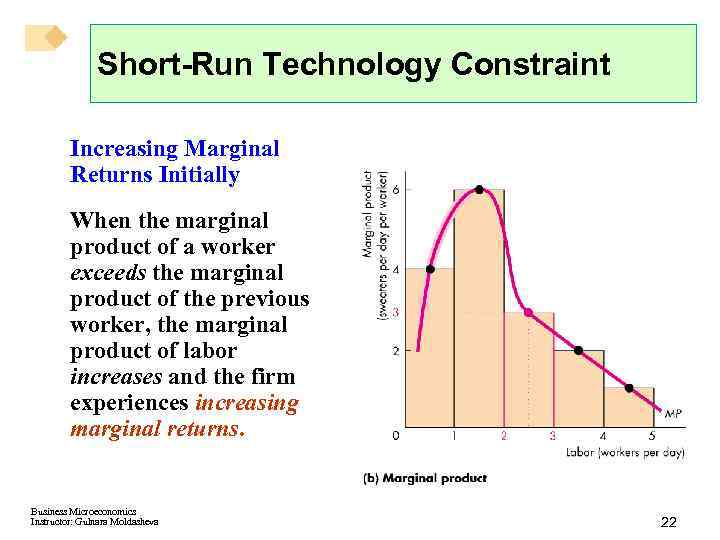Short-Run Technology Constraint Increasing Marginal Returns Initially When the marginal product of a worker exceeds the marginal product of the previous worker, the marginal product of labor increases and the firm experiences increasing marginal returns. Business Microeconomics Instructor: Gulnara Moldasheva 22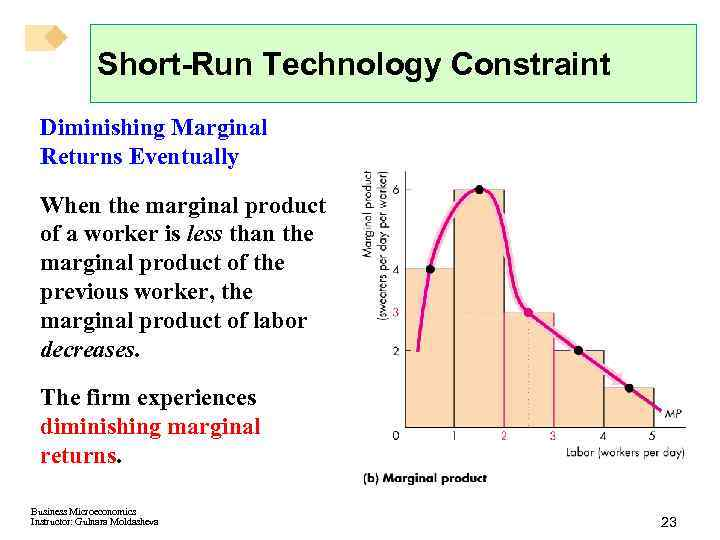Short-Run Technology Constraint Diminishing Marginal Returns Eventually When the marginal product of a worker is less than the marginal product of the previous worker, the marginal product of labor decreases. The firm experiences diminishing marginal returns. Business Microeconomics Instructor: Gulnara Moldasheva 23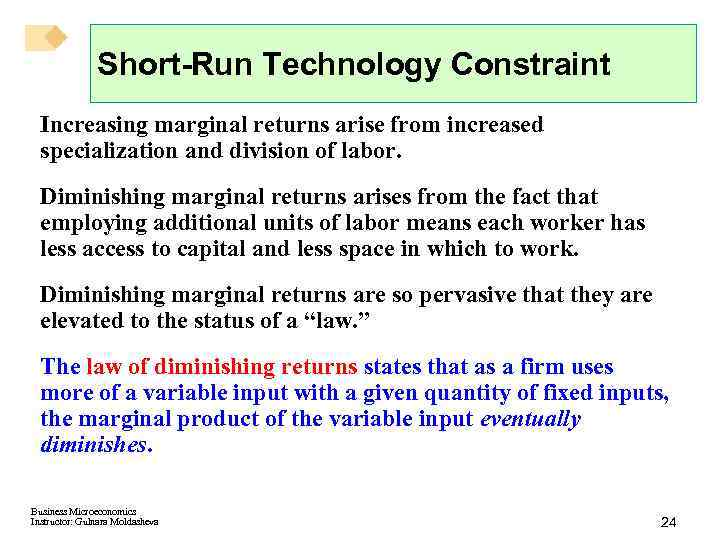Short-Run Technology Constraint Increasing marginal returns arise from increased specialization and division of labor. Diminishing marginal returns arises from the fact that employing additional units of labor means each worker has less access to capital and less space in which to work. Diminishing marginal returns are so pervasive that they are elevated to the status of a “law. ” The law of diminishing returns states that as a firm uses more of a variable input with a given quantity of fixed inputs, the marginal product of the variable input eventually diminishes. Business Microeconomics Instructor: Gulnara Moldasheva 24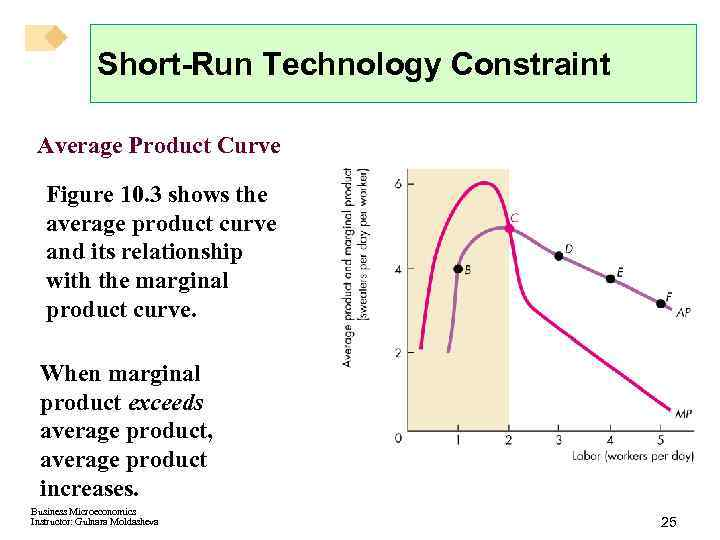Short-Run Technology Constraint Average Product Curve Figure 10. 3 shows the average product curve and its relationship with the marginal product curve. When marginal product exceeds average product, average product increases. Business Microeconomics Instructor: Gulnara Moldasheva 25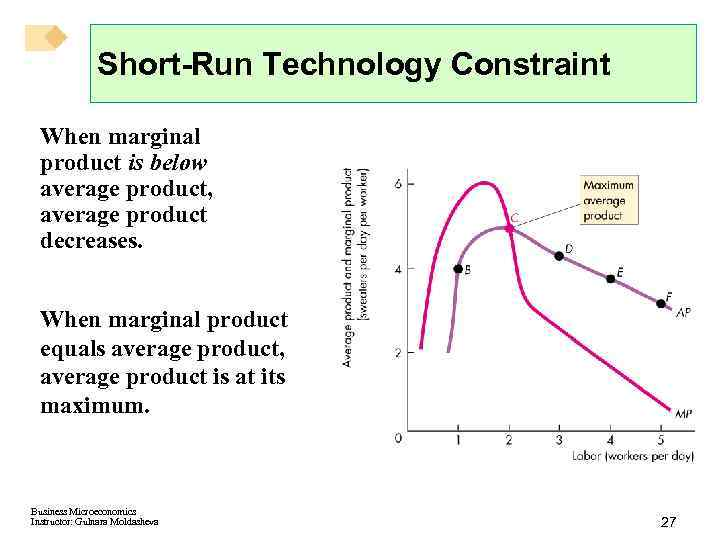Short-Run Technology Constraint When marginal product is below average product, average product decreases. When marginal product equals average product, average product is at its maximum. Business Microeconomics Instructor: Gulnara Moldasheva 27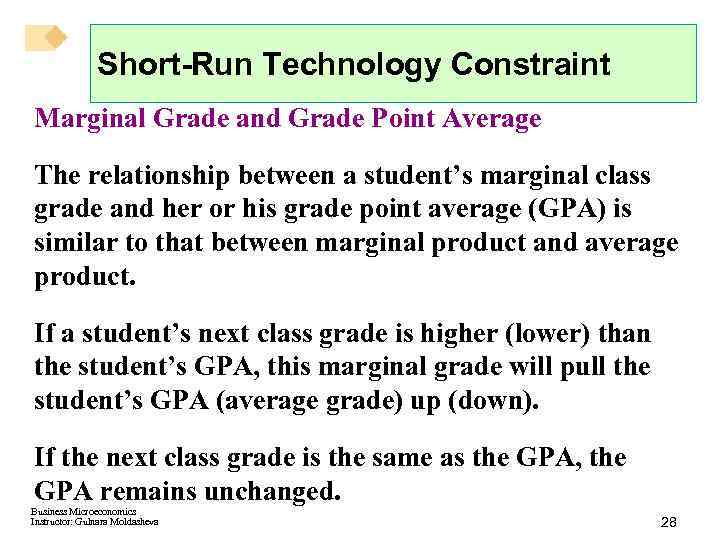Short-Run Technology Constraint Marginal Grade and Grade Point Average The relationship between a student’s marginal class grade and her or his grade point average (GPA) is similar to that between marginal product and average product. If a student’s next class grade is higher (lower) than the student’s GPA, this marginal grade will pull the student’s GPA (average grade) up (down). If the next class grade is the same as the GPA, the GPA remains unchanged. Business Microeconomics Instructor: Gulnara Moldasheva 28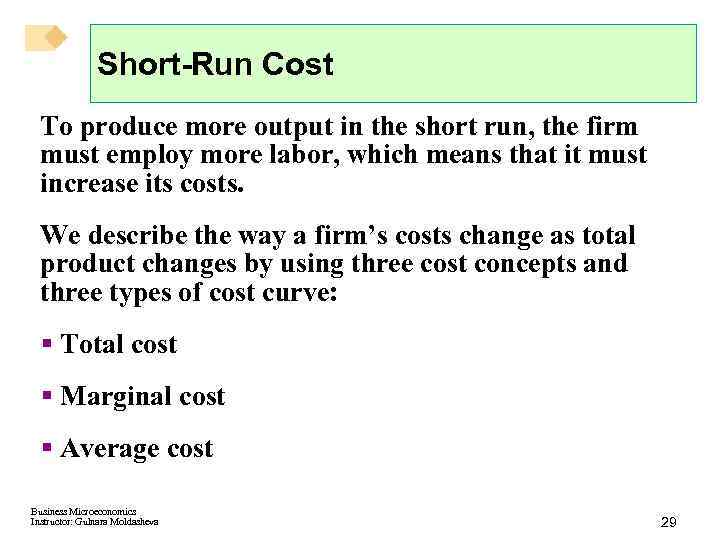Short-Run Cost To produce more output in the short run, the firm must employ more labor, which means that it must increase its costs. We describe the way a firm’s costs change as total product changes by using three cost concepts and three types of cost curve: § Total cost § Marginal cost § Average cost Business Microeconomics Instructor: Gulnara Moldasheva 29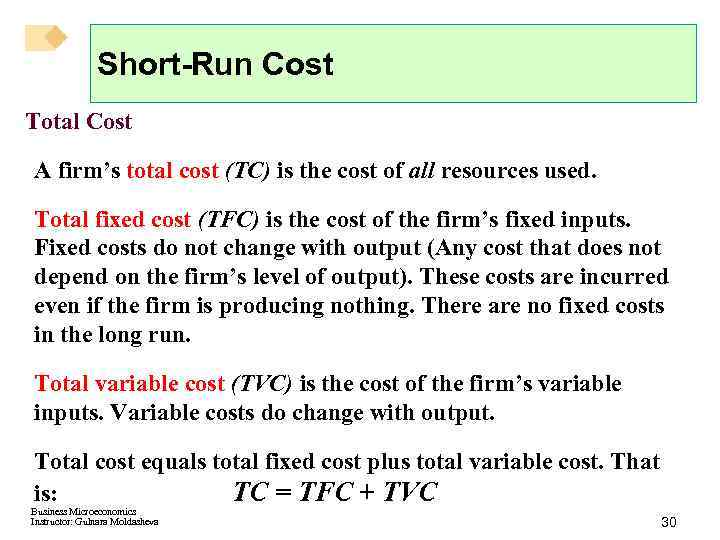Short-Run Cost Total Cost A firm’s total cost (TC) is the cost of all resources used. Total fixed cost (TFC) is the cost of the firm’s fixed inputs. Fixed costs do not change with output (Any cost that does not depend on the firm’s level of output). These costs are incurred even if the firm is producing nothing. There are no fixed costs in the long run. Total variable cost (TVC) is the cost of the firm’s variable inputs. Variable costs do change with output. Total cost equals total fixed cost plus total variable cost. That is: TC = TFC + TVC Business Microeconomics Instructor: Gulnara Moldasheva 30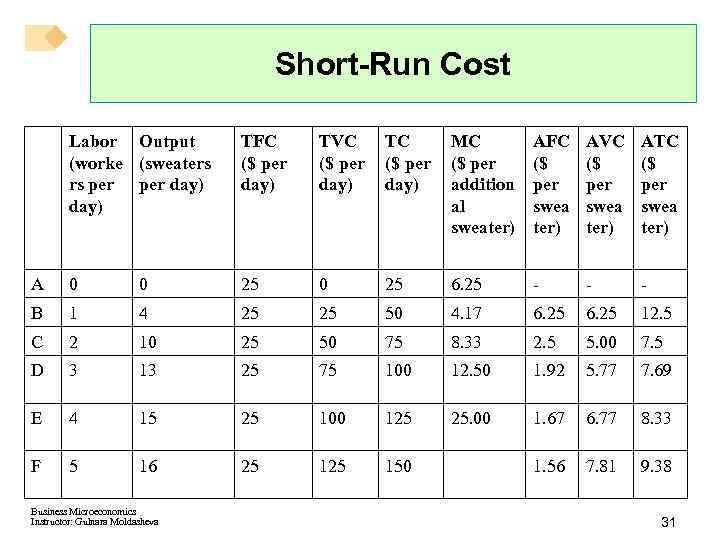Short-Run Cost Labor Output (worke (sweaters rs per day) TFC (\$ per day) TVC (\$ per day) TC (\$ per day) MC (\$ per addition al sweater) AFC (\$ per swea ter) AVC (\$ per swea ter) ATC (\$ per swea ter) A 0 0 25 6. 25 - - - B 1 4 25 25 50 4. 17 6. 25 12. 5 C 2 10 25 50 75 8. 33 2. 5 5. 00 7. 5 D 3 13 25 75 100 12. 50 1. 92 5. 77 7. 69 E 4 15 25 100 125 25. 00 1. 67 6. 77 8. 33 F 5 16 25 150 1. 56 7. 81 9. 38 Business Microeconomics Instructor: Gulnara Moldasheva 31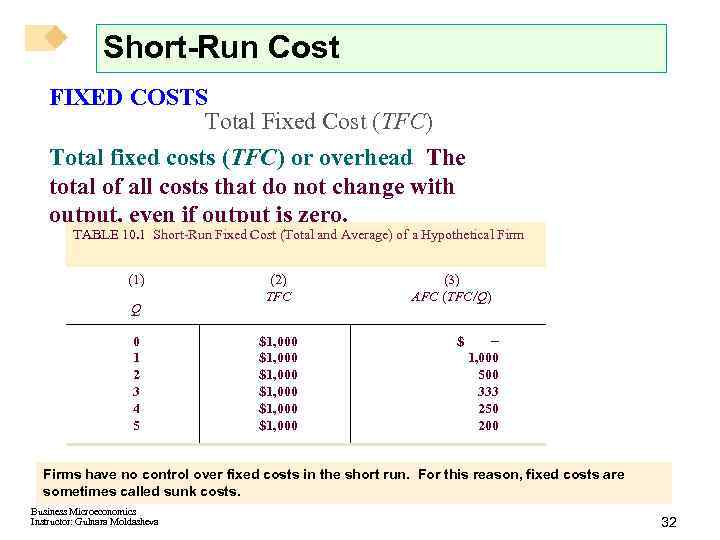Short-Run Cost FIXED COSTS Total Fixed Cost (TFC) Total fixed costs (TFC) or overhead The total of all costs that do not change with output, even if output is zero. TABLE 10. 1 Short-Run Fixed Cost (Total and Average) of a Hypothetical Firm (1) Q 0 1 2 3 4 5 (2) TFC \$1, 000 \$1, 000 (3) AFC (TFC/Q) \$ 1, 000 500 333 250 200 Firms have no control over fixed costs in the short run. For this reason, fixed costs are sometimes called sunk costs. Business Microeconomics Instructor: Gulnara Moldasheva 32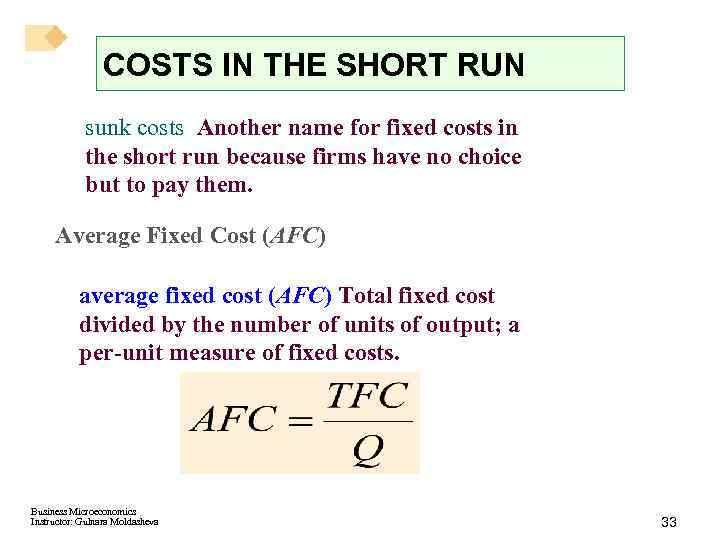COSTS IN THE SHORT RUN sunk costs Another name for fixed costs in the short run because firms have no choice but to pay them. Average Fixed Cost (AFC) average fixed cost (AFC) Total fixed cost divided by the number of units of output; a per-unit measure of fixed costs. Business Microeconomics Instructor: Gulnara Moldasheva 33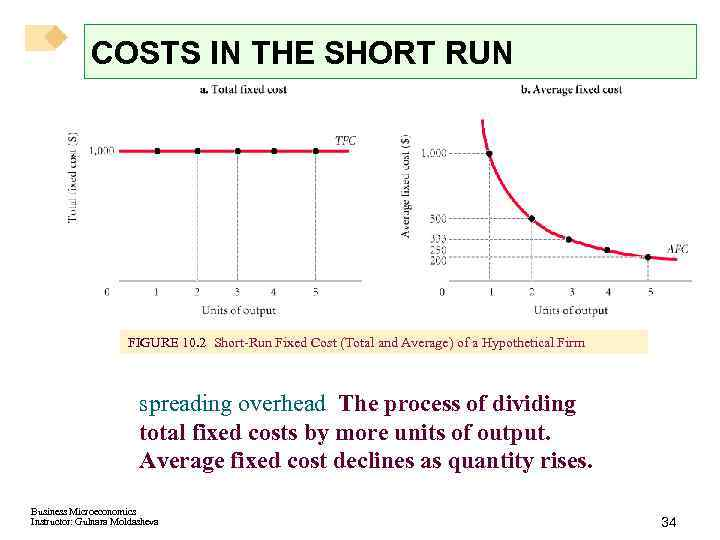COSTS IN THE SHORT RUN FIGURE 10. 2 Short-Run Fixed Cost (Total and Average) of a Hypothetical Firm spreading overhead The process of dividing total fixed costs by more units of output. Average fixed cost declines as quantity rises. Business Microeconomics Instructor: Gulnara Moldasheva 34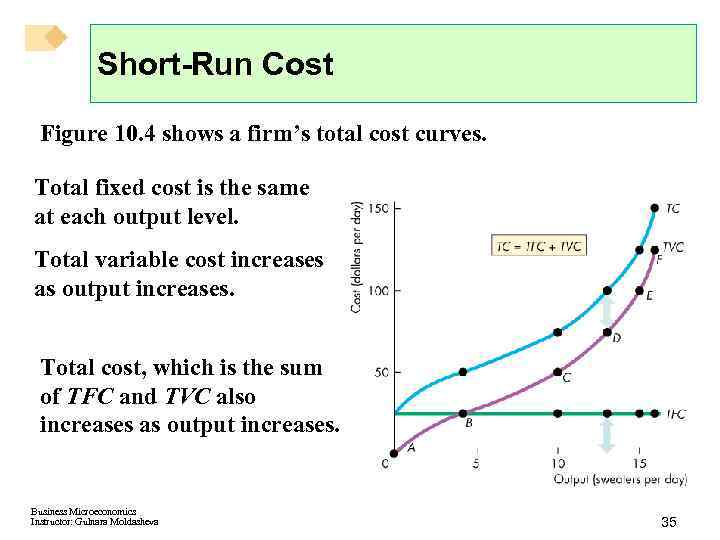Short-Run Cost Figure 10. 4 shows a firm’s total cost curves. Total fixed cost is the same at each output level. Total variable cost increases as output increases. Total cost, which is the sum of TFC and TVC also increases as output increases. Business Microeconomics Instructor: Gulnara Moldasheva 35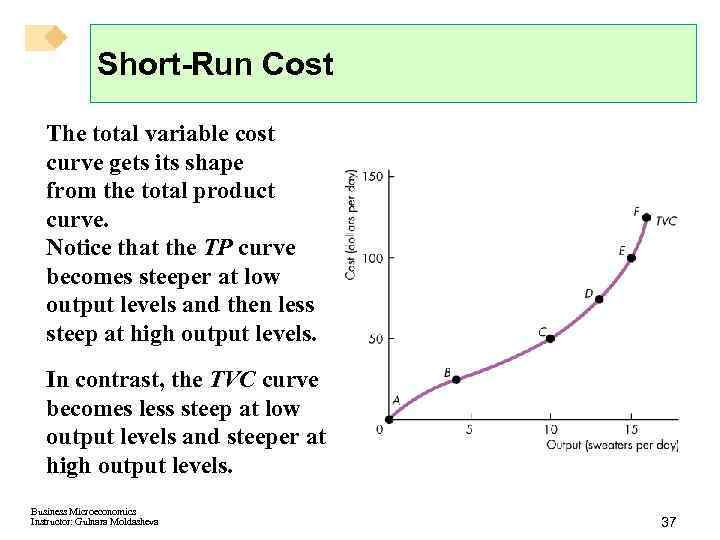Short-Run Cost The total variable cost curve gets its shape from the total product curve. Notice that the TP curve becomes steeper at low output levels and then less steep at high output levels. In contrast, the TVC curve becomes less steep at low output levels and steeper at high output levels. Business Microeconomics Instructor: Gulnara Moldasheva 37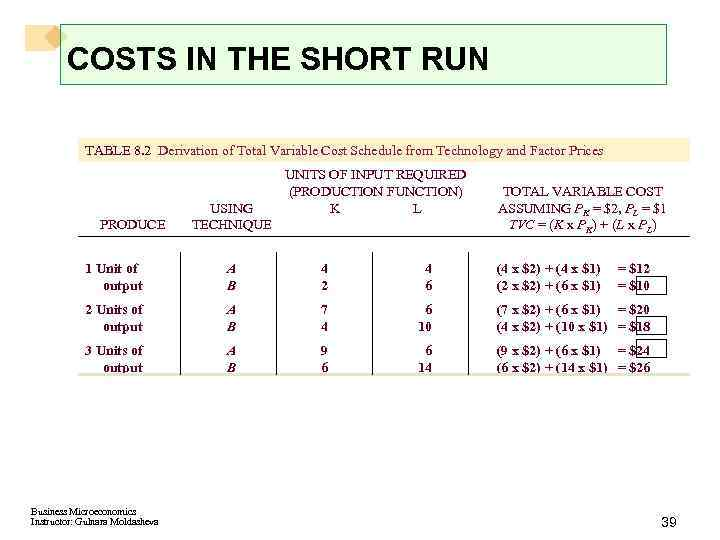COSTS IN THE SHORT RUN TABLE 8. 2 Derivation of Total Variable Cost Schedule from Technology and Factor Prices PRODUCE USING TECHNIQUE UNITS OF INPUT REQUIRED (PRODUCTION FUNCTION) K L TOTAL VARIABLE COST ASSUMING PK = \$2, PL = \$1 TVC = (K x PK) + (L x PL) 1 Unit of output A B 4 2 4 6 2 Units of output A B 7 4 6 10 (7 x \$2) + (6 x \$1) = \$20 (4 x \$2) + (10 x \$1) = \$18 3 Units of output A B 9 6 6 14 (9 x \$2) + (6 x \$1) = \$24 (6 x \$2) + (14 x \$1) = \$26 Business Microeconomics Instructor: Gulnara Moldasheva (4 x \$2) + (4 x \$1) (2 x \$2) + (6 x \$1) = \$12 = \$10 39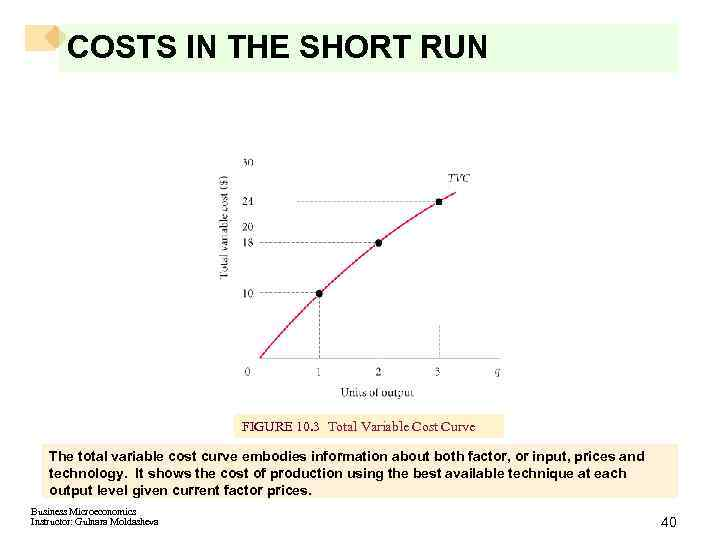COSTS IN THE SHORT RUN FIGURE 10. 3 Total Variable Cost Curve The total variable cost curve embodies information about both factor, or input, prices and technology. It shows the cost of production using the best available technique at each output level given current factor prices. Business Microeconomics Instructor: Gulnara Moldasheva 40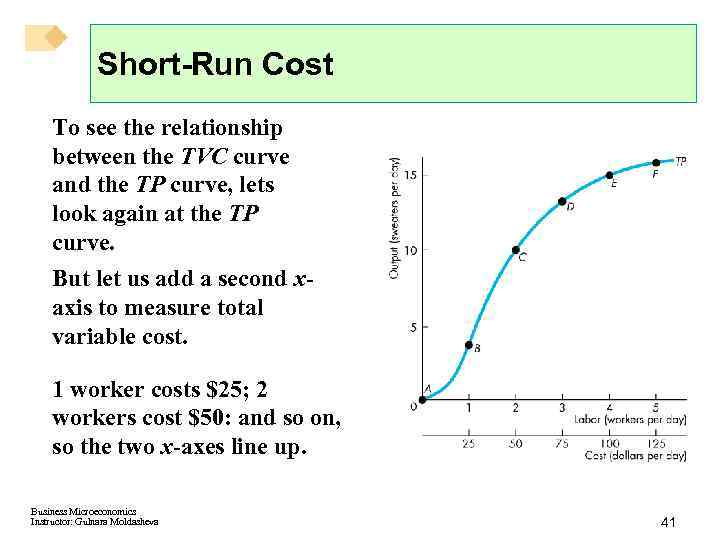Short-Run Cost To see the relationship between the TVC curve and the TP curve, lets look again at the TP curve. But let us add a second xaxis to measure total variable cost. 1 worker costs \$25; 2 workers cost \$50: and so on, so the two x-axes line up. Business Microeconomics Instructor: Gulnara Moldasheva 41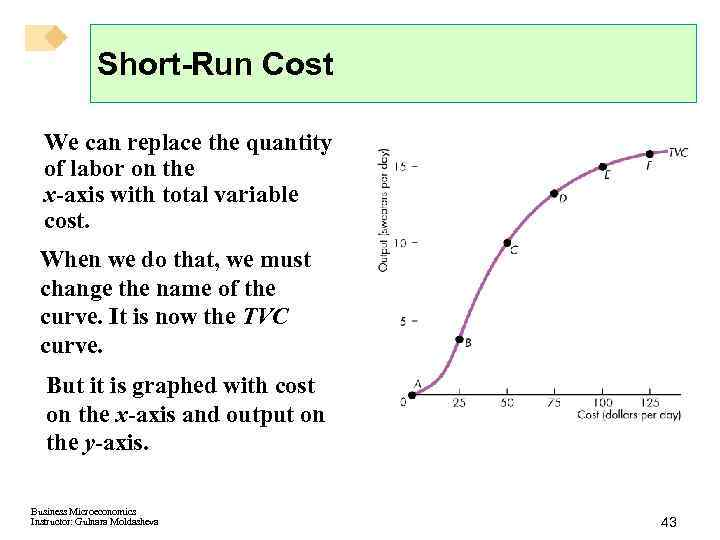Short-Run Cost We can replace the quantity of labor on the x-axis with total variable cost. When we do that, we must change the name of the curve. It is now the TVC curve. But it is graphed with cost on the x-axis and output on the y-axis. Business Microeconomics Instructor: Gulnara Moldasheva 43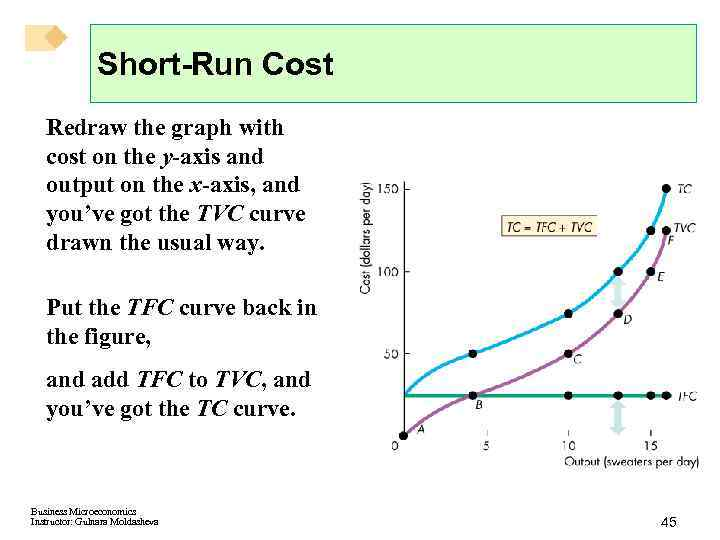Short-Run Cost Redraw the graph with cost on the y-axis and output on the x-axis, and you’ve got the TVC curve drawn the usual way. Put the TFC curve back in the figure, and add TFC to TVC, and you’ve got the TC curve. Business Microeconomics Instructor: Gulnara Moldasheva 45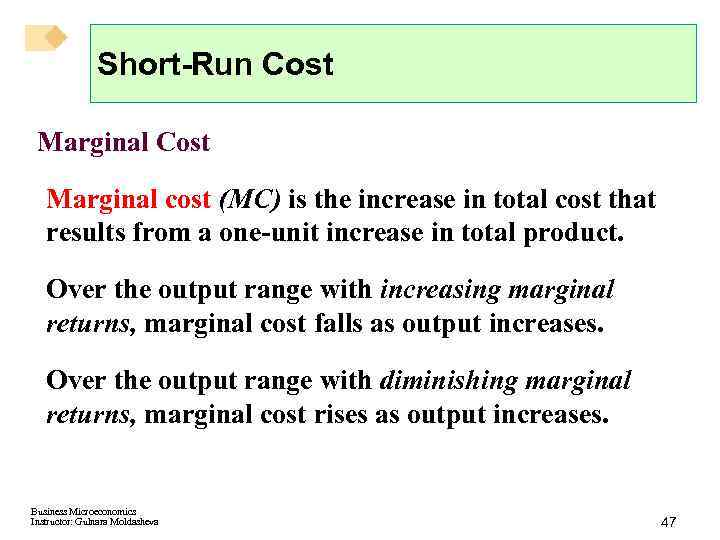Short-Run Cost Marginal cost (MC) is the increase in total cost that results from a one-unit increase in total product. Over the output range with increasing marginal returns, marginal cost falls as output increases. Over the output range with diminishing marginal returns, marginal cost rises as output increases. Business Microeconomics Instructor: Gulnara Moldasheva 47COSTS IN THE SHORT RUN Marginal cost (MC) The increase in total cost that results from producing one more unit of output. Marginal costs reflect changes in variable costs. TABLE 10. 3 Derivation of Marginal Cost from Total Variable Cost UNITS OF OUTPUT TOTAL VARIABLE COSTS (\$) MARGINAL COSTS (\$) 0 0 0 1 10 10 2 18 8 3 24 6 Although the easiest way to derive marginal cost is to look at total variable cost and subtract, do not lose sight of the fact that when a firm increases its output level, it hires or demands more inputs. Marginal cost measures the additional cost of inputs required to produce each successive unit of output. Business Microeconomics Instructor: Gulnara Moldasheva 48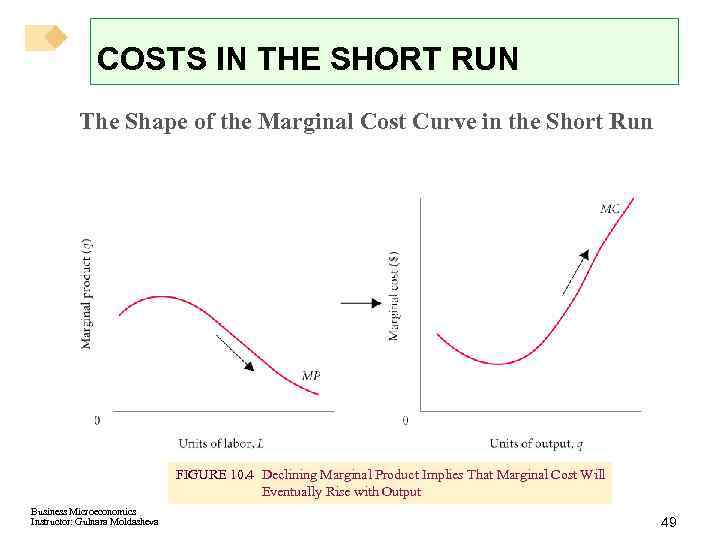COSTS IN THE SHORT RUN The Shape of the Marginal Cost Curve in the Short Run FIGURE 10. 4 Declining Marginal Product Implies That Marginal Cost Will Eventually Rise with Output Business Microeconomics Instructor: Gulnara Moldasheva 49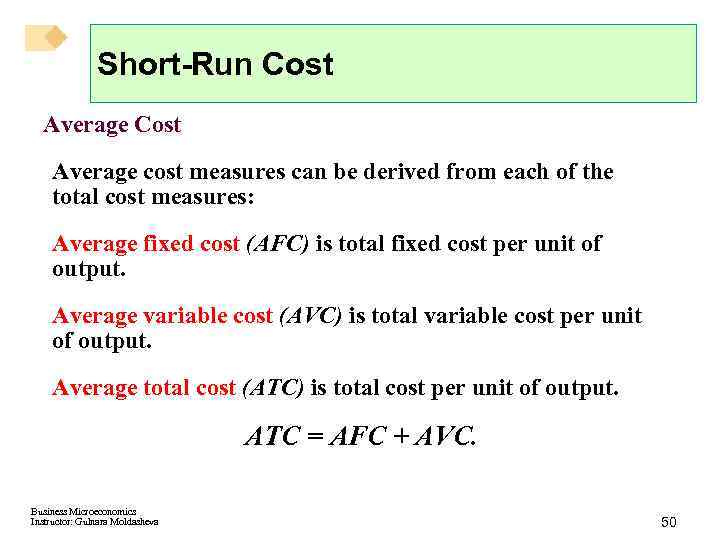Short-Run Cost Average cost measures can be derived from each of the total cost measures: Average fixed cost (AFC) is total fixed cost per unit of output. Average variable cost (AVC) is total variable cost per unit of output. Average total cost (ATC) is total cost per unit of output. ATC = AFC + AVC. Business Microeconomics Instructor: Gulnara Moldasheva 50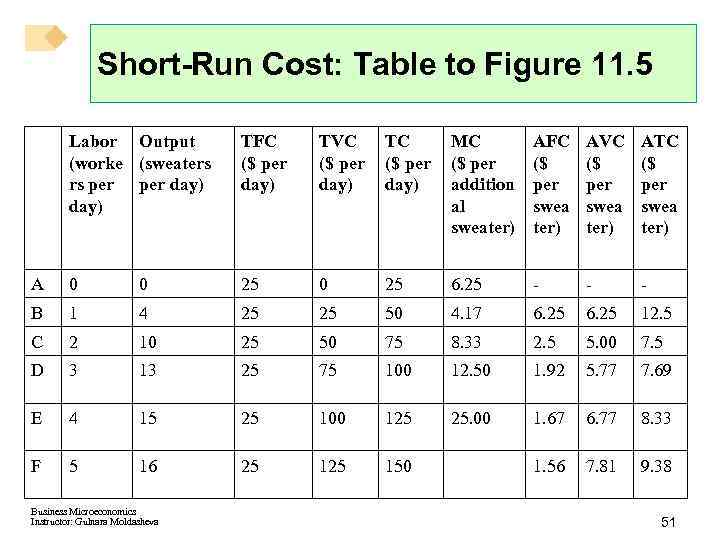Short-Run Cost: Table to Figure 11. 5 Labor Output (worke (sweaters rs per day) TFC (\$ per day) TVC (\$ per day) TC (\$ per day) MC (\$ per addition al sweater) AFC (\$ per swea ter) AVC (\$ per swea ter) ATC (\$ per swea ter) A 0 0 25 6. 25 - - - B 1 4 25 25 50 4. 17 6. 25 12. 5 C 2 10 25 50 75 8. 33 2. 5 5. 00 7. 5 D 3 13 25 75 100 12. 50 1. 92 5. 77 7. 69 E 4 15 25 100 125 25. 00 1. 67 6. 77 8. 33 F 5 16 25 150 1. 56 7. 81 9. 38 Business Microeconomics Instructor: Gulnara Moldasheva 51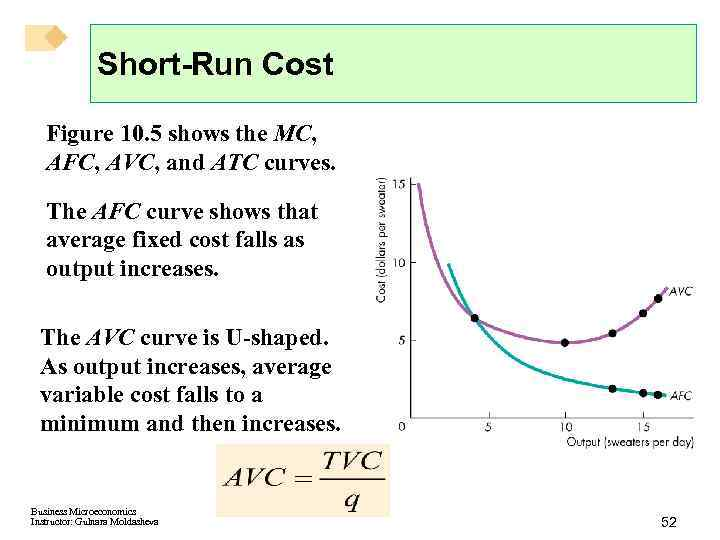Short-Run Cost Figure 10. 5 shows the MC, AFC, AVC, and ATC curves. The AFC curve shows that average fixed cost falls as output increases. The AVC curve is U-shaped. As output increases, average variable cost falls to a minimum and then increases. Business Microeconomics Instructor: Gulnara Moldasheva 52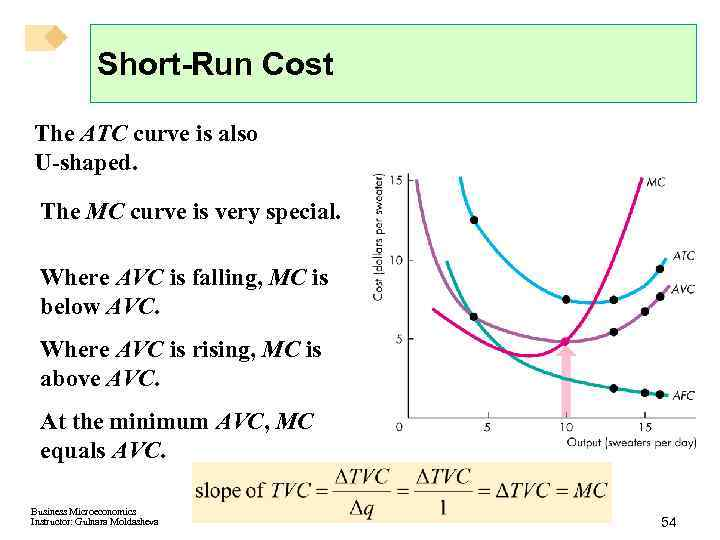Short-Run Cost The ATC curve is also U-shaped. The MC curve is very special. Where AVC is falling, MC is below AVC. Where AVC is rising, MC is above AVC. At the minimum AVC, MC equals AVC. Business Microeconomics Instructor: Gulnara Moldasheva 54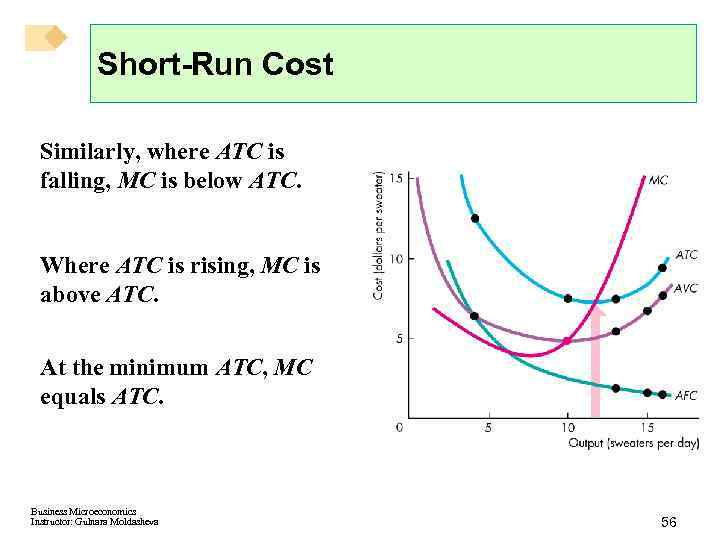Short-Run Cost Similarly, where ATC is falling, MC is below ATC. Where ATC is rising, MC is above ATC. At the minimum ATC, MC equals ATC. Business Microeconomics Instructor: Gulnara Moldasheva 56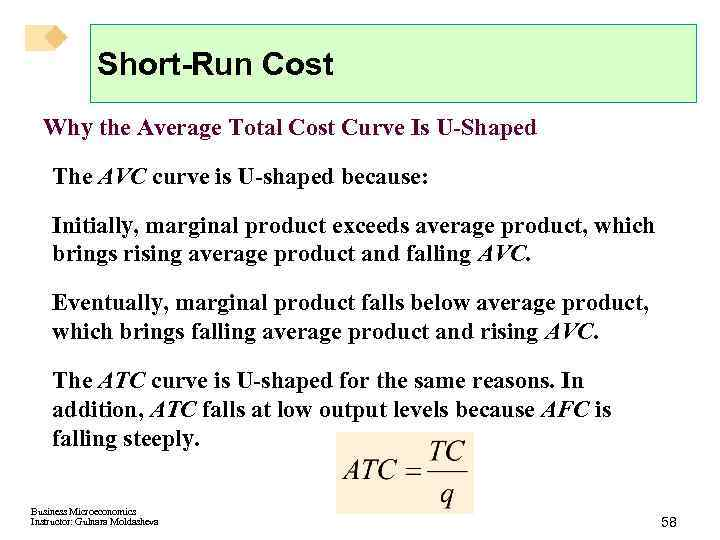Short-Run Cost Why the Average Total Cost Curve Is U-Shaped The AVC curve is U-shaped because: Initially, marginal product exceeds average product, which brings rising average product and falling AVC. Eventually, marginal product falls below average product, which brings falling average product and rising AVC. The ATC curve is U-shaped for the same reasons. In addition, ATC falls at low output levels because AFC is falling steeply. Business Microeconomics Instructor: Gulnara Moldasheva 58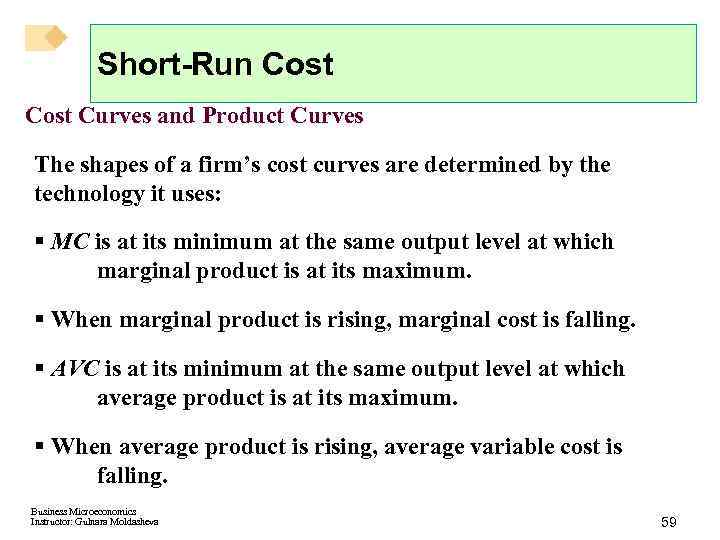Short-Run Cost Curves and Product Curves The shapes of a firm’s cost curves are determined by the technology it uses: § MC is at its minimum at the same output level at which marginal product is at its maximum. § When marginal product is rising, marginal cost is falling. § AVC is at its minimum at the same output level at which average product is at its maximum. § When average product is rising, average variable cost is falling. Business Microeconomics Instructor: Gulnara Moldasheva 59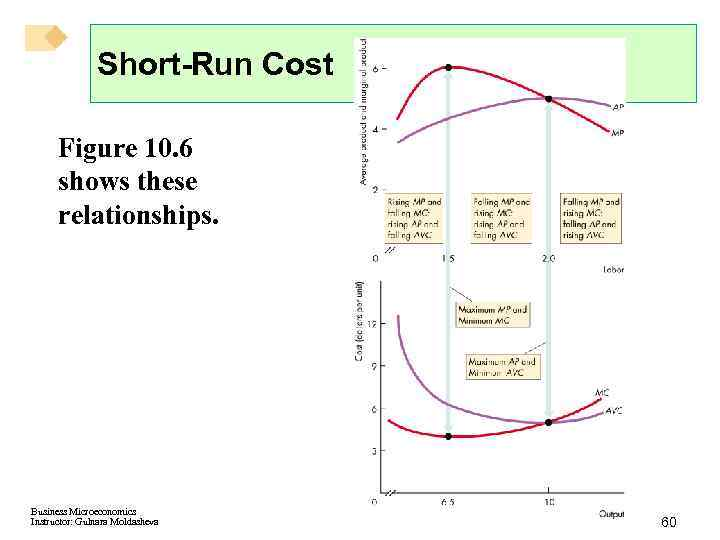Short-Run Cost Figure 10. 6 shows these relationships. Business Microeconomics Instructor: Gulnara Moldasheva 60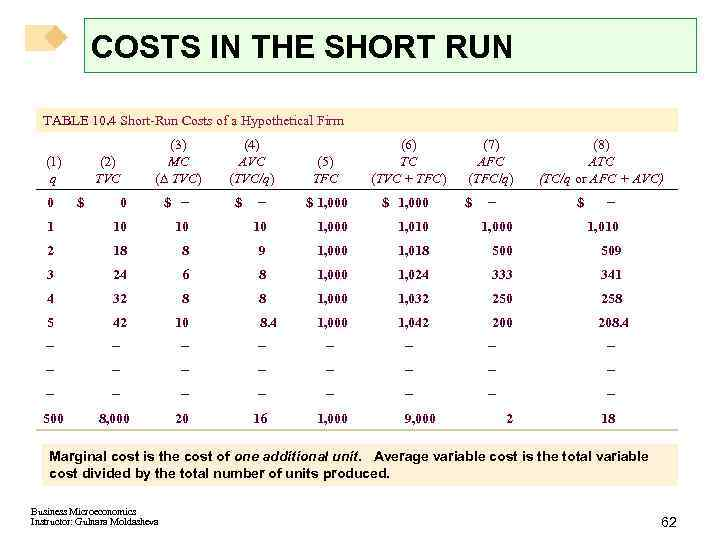COSTS IN THE SHORT RUN TABLE 10. 4 Short-Run Costs of a Hypothetical Firm (1) q (2) TVC (3) MC (D TVC) (4) AVC (TVC/q) (5) TFC (6) TC (TVC + TFC) - \$ 1, 000 (7) AFC (TFC/q) 0 \$ - 1 10 10 10 1, 000 1, 010 2 18 8 9 1, 000 1, 018 500 509 3 24 6 8 1, 000 1, 024 333 341 4 32 8 8 1, 000 1, 032 250 258 5 42 10 8. 4 1, 000 1, 042 200 208. 4 - - - - - - 500 8, 000 20 16 1, 000 0 \$ \$ 9, 000 \$ - (8) ATC (TC/q or AFC + AVC) \$ 2 - 18 Marginal cost is the cost of one additional unit. Average variable cost is the total variable cost divided by the total number of units produced. Business Microeconomics Instructor: Gulnara Moldasheva 62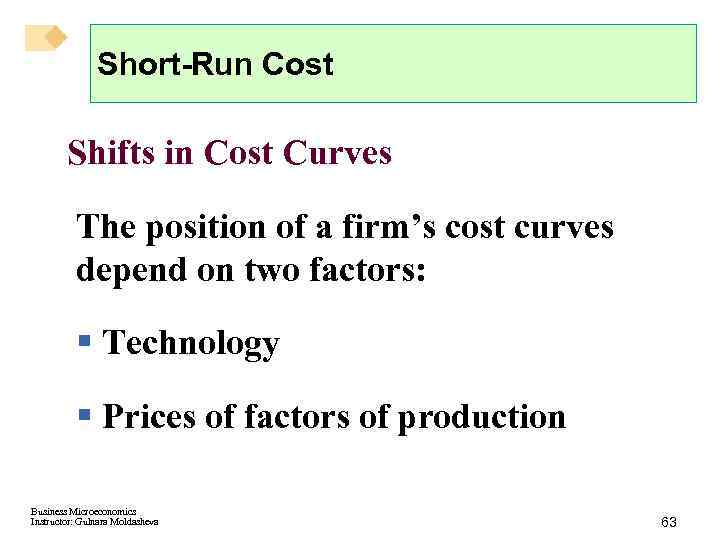Short-Run Cost Shifts in Cost Curves The position of a firm’s cost curves depend on two factors: § Technology § Prices of factors of production Business Microeconomics Instructor: Gulnara Moldasheva 63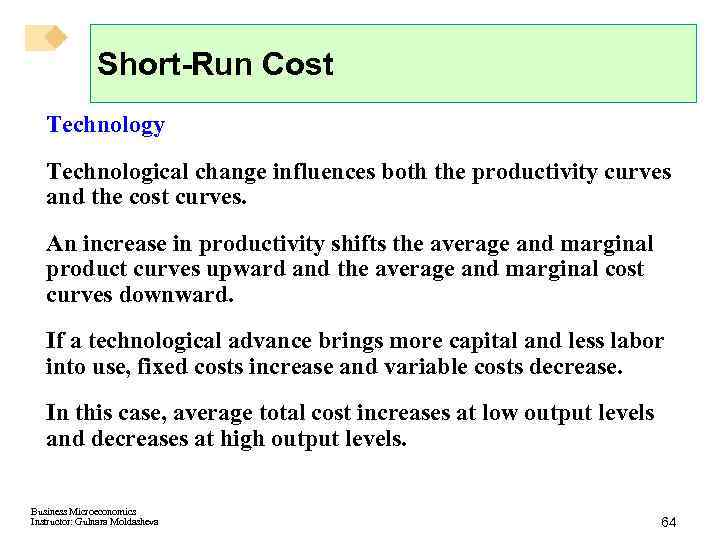Short-Run Cost Technology Technological change influences both the productivity curves and the cost curves. An increase in productivity shifts the average and marginal product curves upward and the average and marginal cost curves downward. If a technological advance brings more capital and less labor into use, fixed costs increase and variable costs decrease. In this case, average total cost increases at low output levels and decreases at high output levels. Business Microeconomics Instructor: Gulnara Moldasheva 64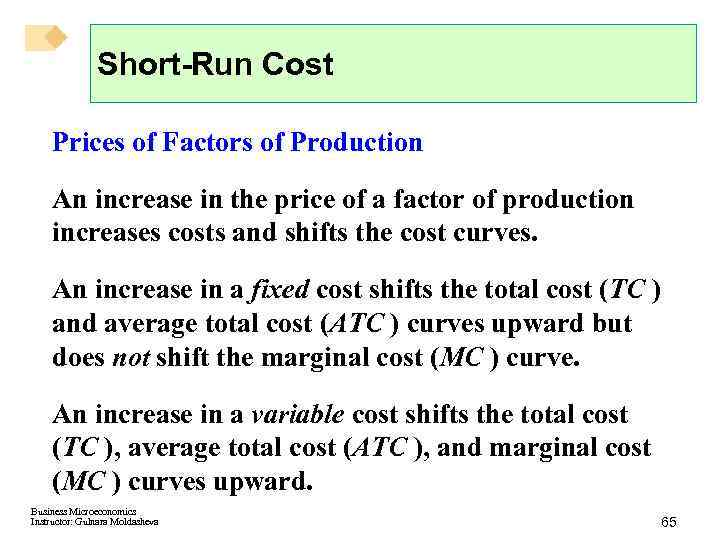Short-Run Cost Prices of Factors of Production An increase in the price of a factor of production increases costs and shifts the cost curves. An increase in a fixed cost shifts the total cost (TC ) and average total cost (ATC ) curves upward but does not shift the marginal cost (MC ) curve. An increase in a variable cost shifts the total cost (TC ), average total cost (ATC ), and marginal cost (MC ) curves upward. Business Microeconomics Instructor: Gulnara Moldasheva 65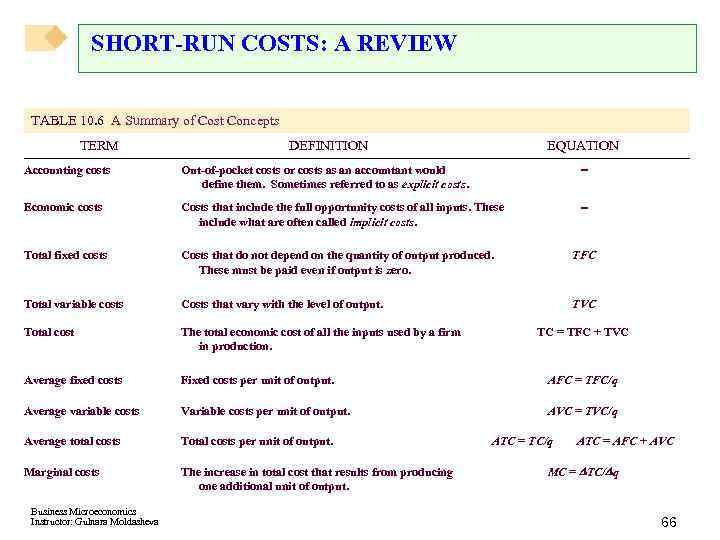SHORT-RUN COSTS: A REVIEW TABLE 10. 6 A Summary of Cost Concepts TERM DEFINITION EQUATION Accounting costs Out-of-pocket costs or costs as an accountant would define them. Sometimes referred to as explicit costs. - Economic costs Costs that include the full opportunity costs of all inputs. These include what are often called implicit costs. - Total fixed costs Costs that do not depend on the quantity of output produced. These must be paid even if output is zero. TFC Total variable costs Costs that vary with the level of output. TVC Total cost The total economic cost of all the inputs used by a firm in production. Average fixed costs Fixed costs per unit of output. AFC = TFC/q Average variable costs Variable costs per unit of output. AVC = TVC/q Average total costs Total costs per unit of output. Marginal costs The increase in total cost that results from producing one additional unit of output. Business Microeconomics Instructor: Gulnara Moldasheva TC = TFC + TVC ATC = TC/q ATC = AFC + AVC MC = DTC/Dq 66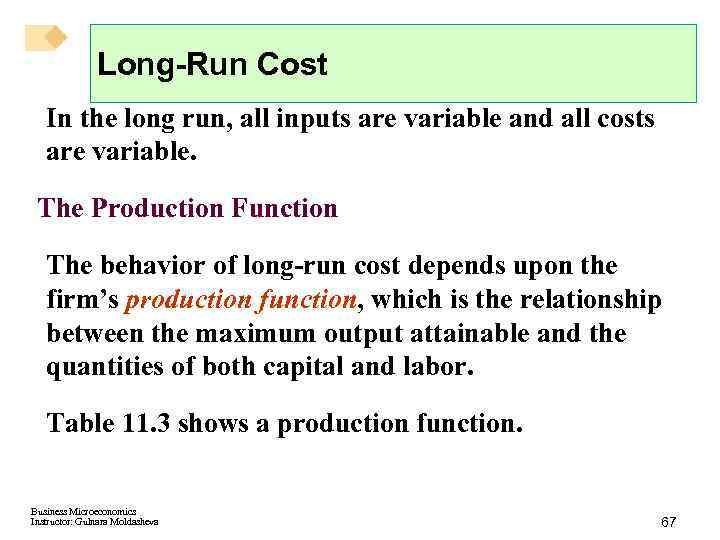Long-Run Cost In the long run, all inputs are variable and all costs are variable. The Production Function The behavior of long-run cost depends upon the firm’s production function, which is the relationship between the maximum output attainable and the quantities of both capital and labor. Table 11. 3 shows a production function. Business Microeconomics Instructor: Gulnara Moldasheva 67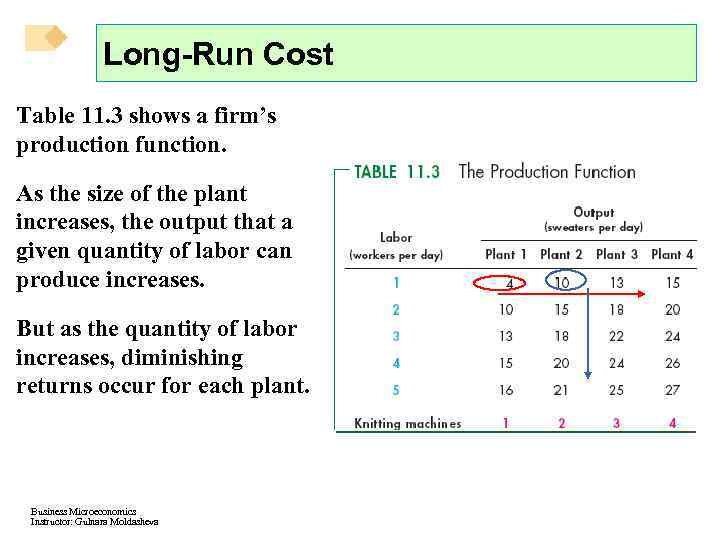Long-Run Cost Table 11. 3 shows a firm’s production function. As the size of the plant increases, the output that a given quantity of labor can produce increases. But as the quantity of labor increases, diminishing returns occur for each plant. Business Microeconomics Instructor: Gulnara Moldasheva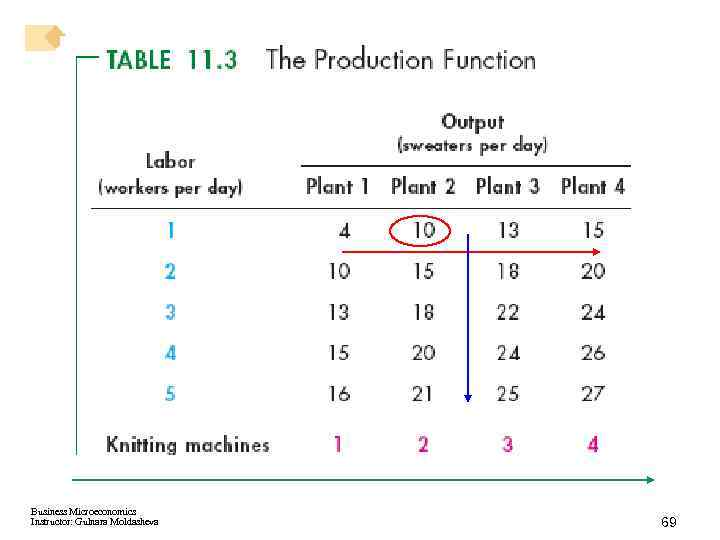Business Microeconomics Instructor: Gulnara Moldasheva 69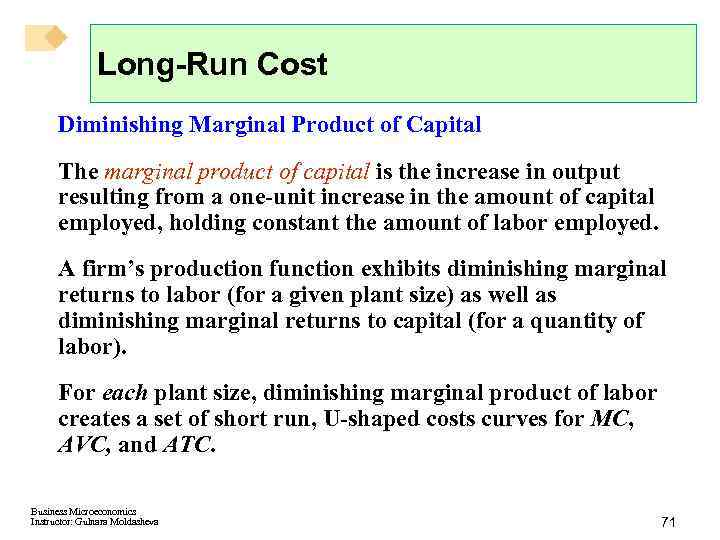Long-Run Cost Diminishing Marginal Product of Capital The marginal product of capital is the increase in output resulting from a one-unit increase in the amount of capital employed, holding constant the amount of labor employed. A firm’s production function exhibits diminishing marginal returns to labor (for a given plant size) as well as diminishing marginal returns to capital (for a quantity of labor). For each plant size, diminishing marginal product of labor creates a set of short run, U-shaped costs curves for MC, AVC, and ATC. Business Microeconomics Instructor: Gulnara Moldasheva 71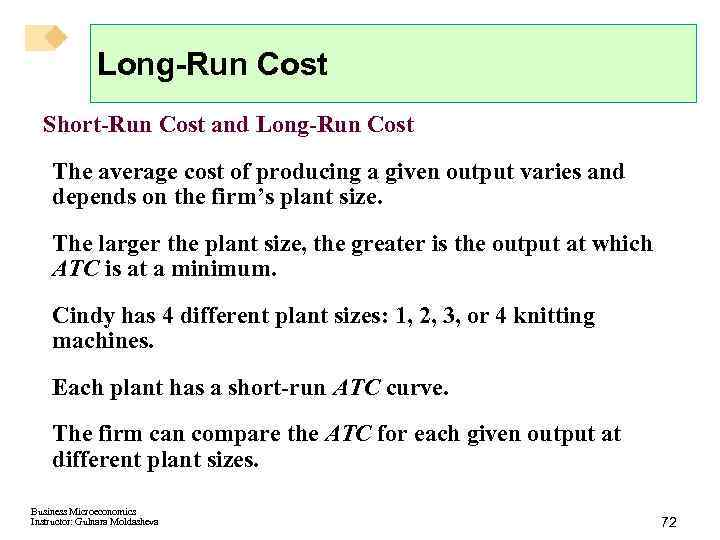Long-Run Cost Short-Run Cost and Long-Run Cost The average cost of producing a given output varies and depends on the firm’s plant size. The larger the plant size, the greater is the output at which ATC is at a minimum. Cindy has 4 different plant sizes: 1, 2, 3, or 4 knitting machines. Each plant has a short-run ATC curve. The firm can compare the ATC for each given output at different plant sizes. Business Microeconomics Instructor: Gulnara Moldasheva 72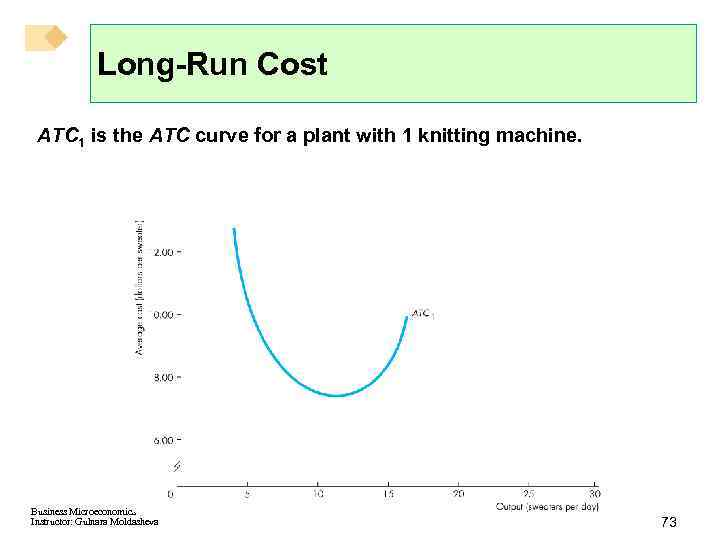Long-Run Cost ATC 1 is the ATC curve for a plant with 1 knitting machine. Business Microeconomics Instructor: Gulnara Moldasheva 73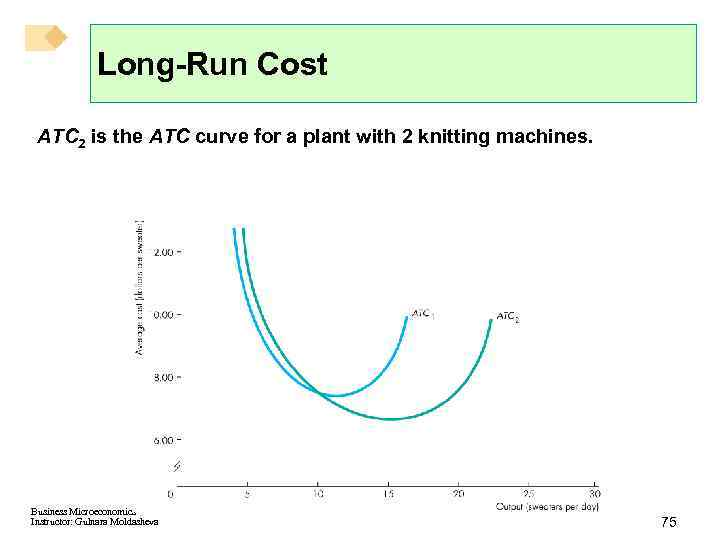Long-Run Cost ATC 2 is the ATC curve for a plant with 2 knitting machines. Business Microeconomics Instructor: Gulnara Moldasheva 75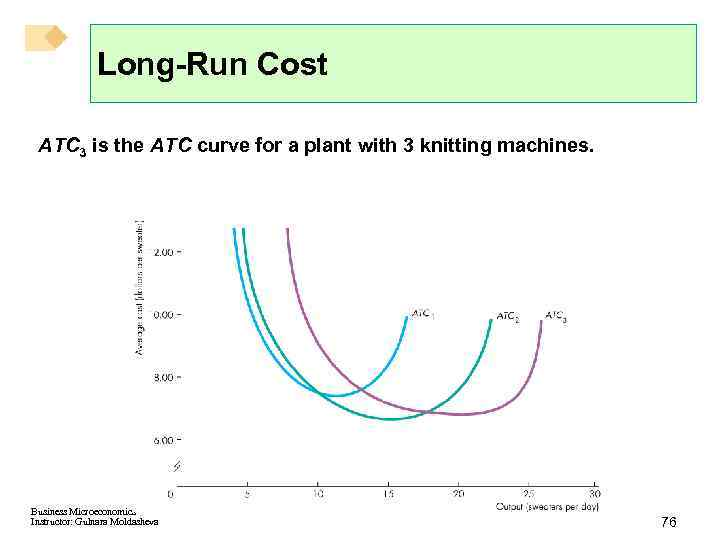Long-Run Cost ATC 3 is the ATC curve for a plant with 3 knitting machines. Business Microeconomics Instructor: Gulnara Moldasheva 76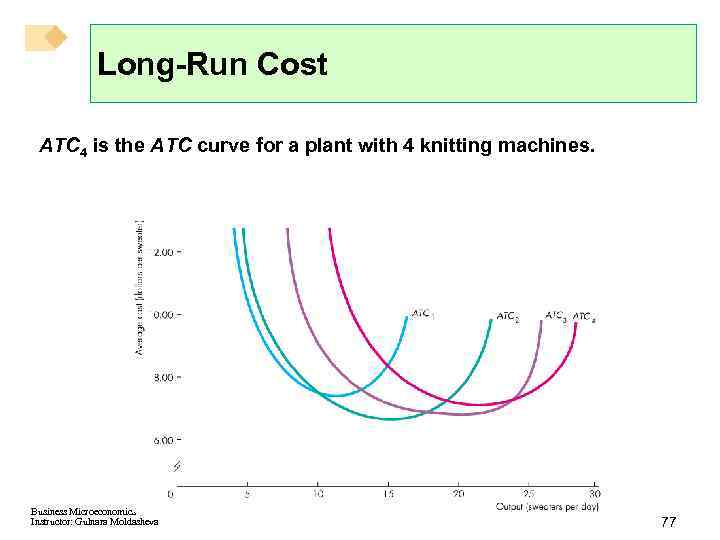Long-Run Cost ATC 4 is the ATC curve for a plant with 4 knitting machines. Business Microeconomics Instructor: Gulnara Moldasheva 77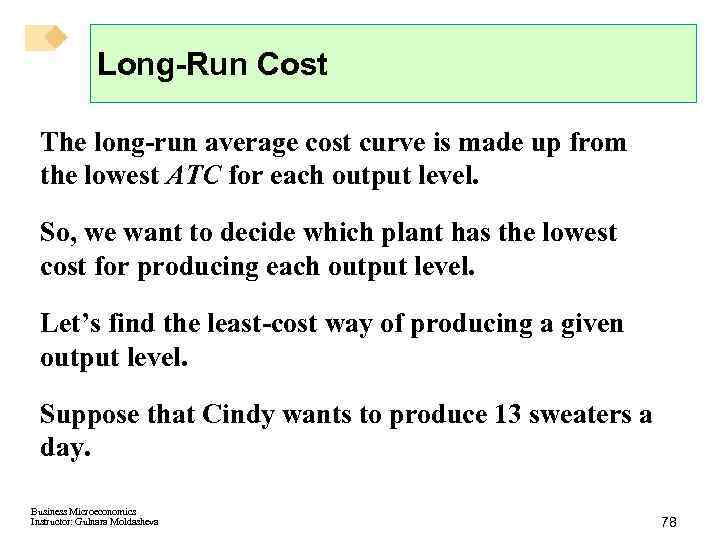Long-Run Cost The long-run average cost curve is made up from the lowest ATC for each output level. So, we want to decide which plant has the lowest cost for producing each output level. Let’s find the least-cost way of producing a given output level. Suppose that Cindy wants to produce 13 sweaters a day. Business Microeconomics Instructor: Gulnara Moldasheva 78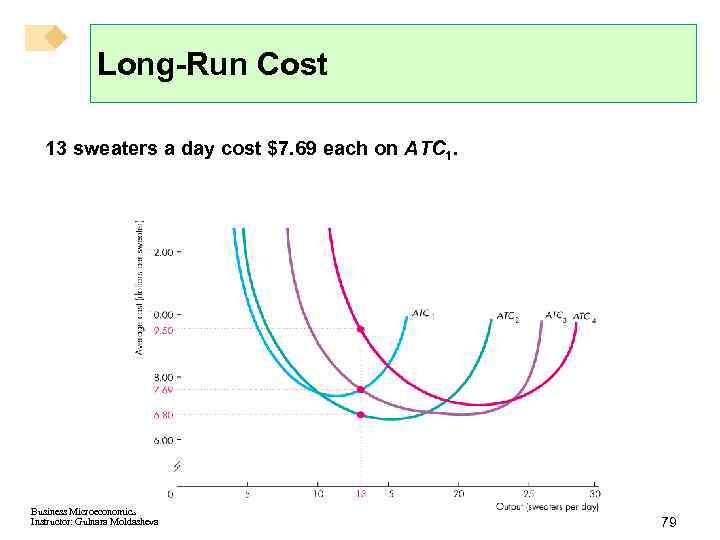Long-Run Cost 13 sweaters a day cost \$7. 69 each on ATC 1. Business Microeconomics Instructor: Gulnara Moldasheva 79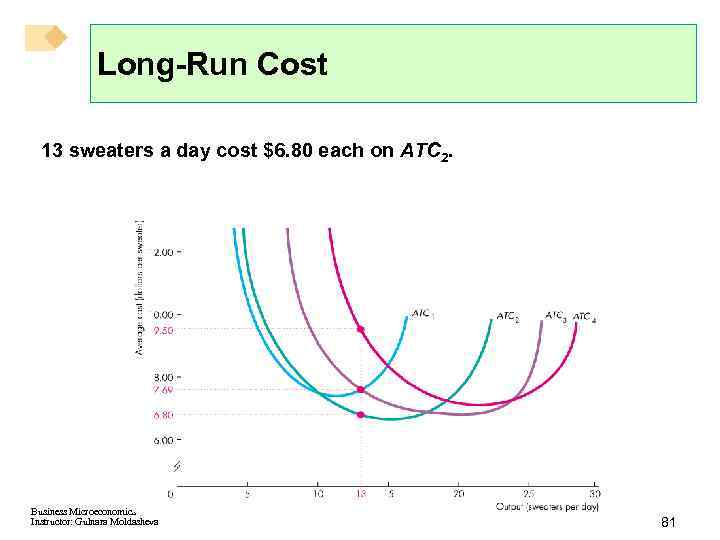Long-Run Cost 13 sweaters a day cost \$6. 80 each on ATC 2. Business Microeconomics Instructor: Gulnara Moldasheva 81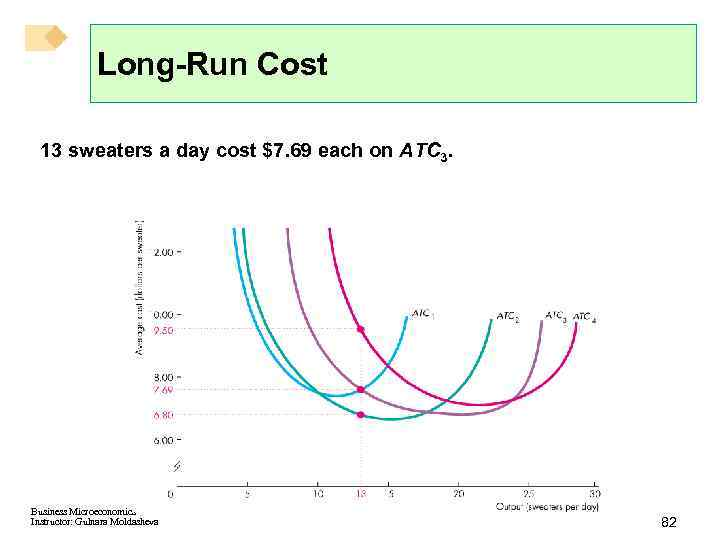Long-Run Cost 13 sweaters a day cost \$7. 69 each on ATC 3. Business Microeconomics Instructor: Gulnara Moldasheva 82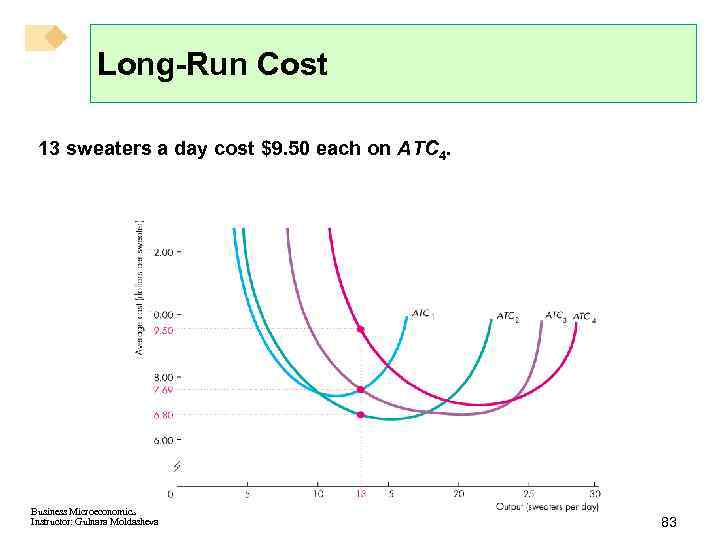Long-Run Cost 13 sweaters a day cost \$9. 50 each on ATC 4. Business Microeconomics Instructor: Gulnara Moldasheva 83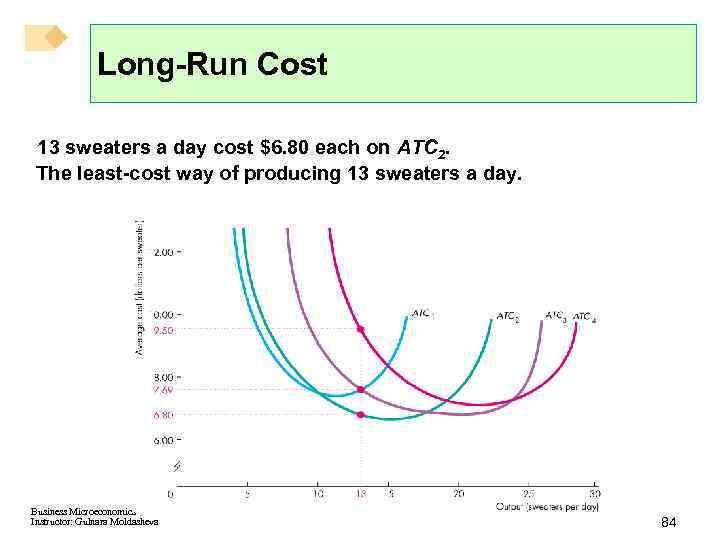Long-Run Cost 13 sweaters a day cost \$6. 80 each on ATC 2. The least-cost way of producing 13 sweaters a day. Business Microeconomics Instructor: Gulnara Moldasheva 84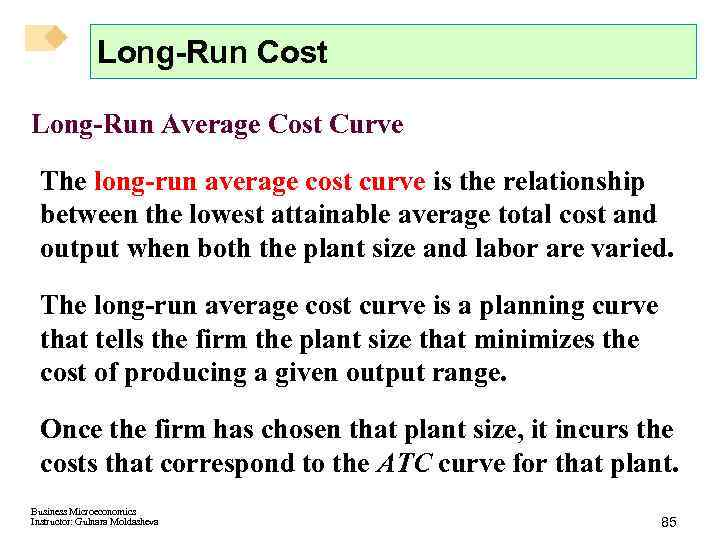Long-Run Cost Long-Run Average Cost Curve The long-run average cost curve is the relationship between the lowest attainable average total cost and output when both the plant size and labor are varied. The long-run average cost curve is a planning curve that tells the firm the plant size that minimizes the cost of producing a given output range. Once the firm has chosen that plant size, it incurs the costs that correspond to the ATC curve for that plant. Business Microeconomics Instructor: Gulnara Moldasheva 85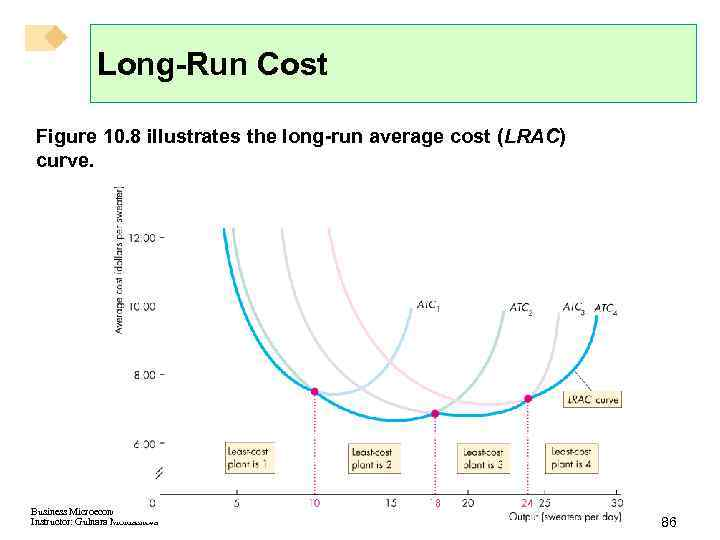Long-Run Cost Figure 10. 8 illustrates the long-run average cost (LRAC) curve. Business Microeconomics Instructor: Gulnara Moldasheva 86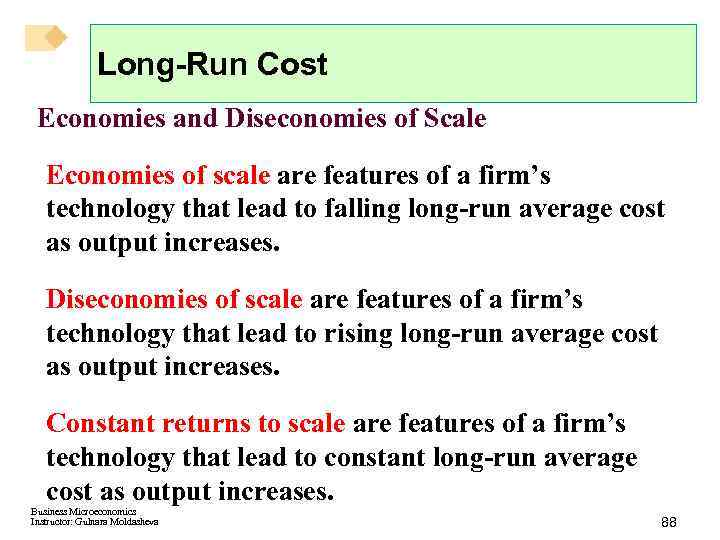Long-Run Cost Economies and Diseconomies of Scale Economies of scale are features of a firm’s technology that lead to falling long-run average cost as output increases. Diseconomies of scale are features of a firm’s technology that lead to rising long-run average cost as output increases. Constant returns to scale are features of a firm’s technology that lead to constant long-run average cost as output increases. Business Microeconomics Instructor: Gulnara Moldasheva 88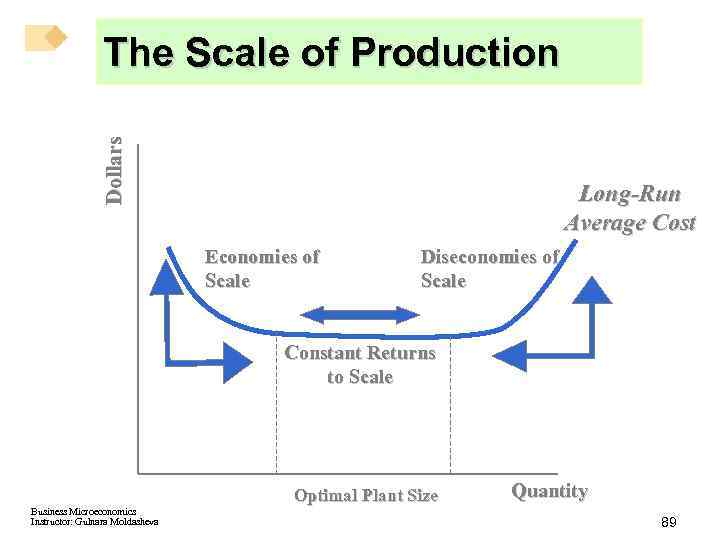Dollars The Scale of Production Long-Run Average Cost Economies of Scale Diseconomies of Scale Constant Returns to Scale Optimal Plant Size Business Microeconomics Instructor: Gulnara Moldasheva Quantity 89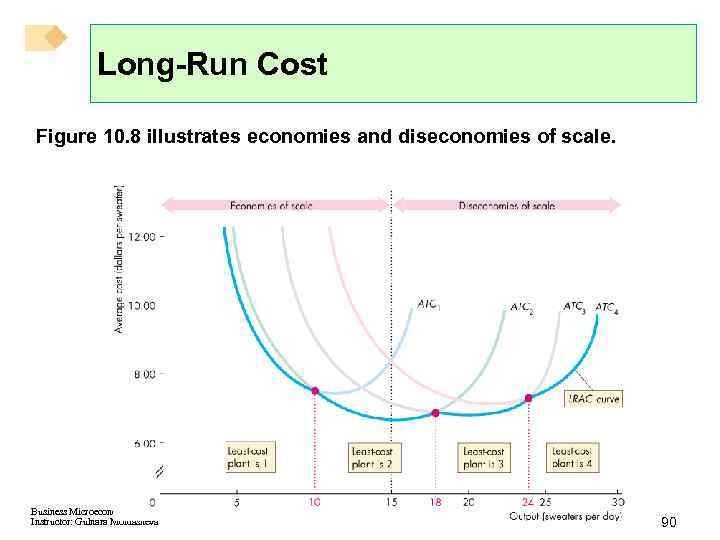Long-Run Cost Figure 10. 8 illustrates economies and diseconomies of scale. Business Microeconomics Instructor: Gulnara Moldasheva 90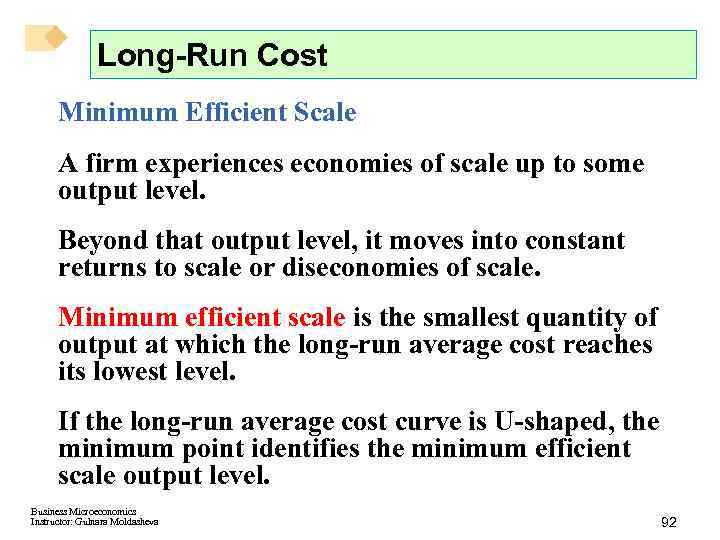Long-Run Cost Minimum Efficient Scale A firm experiences economies of scale up to some output level. Beyond that output level, it moves into constant returns to scale or diseconomies of scale. Minimum efficient scale is the smallest quantity of output at which the long-run average cost reaches its lowest level. If the long-run average cost curve is U-shaped, the minimum point identifies the minimum efficient scale output level. Business Microeconomics Instructor: Gulnara Moldasheva 92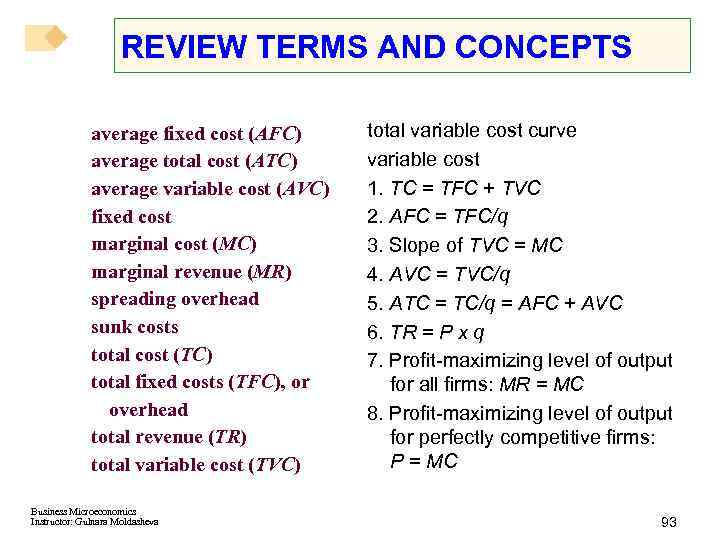REVIEW TERMS AND CONCEPTS average fixed cost (AFC) average total cost (ATC) average variable cost (AVC) fixed cost marginal cost (MC) marginal revenue (MR) spreading overhead sunk costs total cost (TC) total fixed costs (TFC), or overhead total revenue (TR) total variable cost (TVC) Business Microeconomics Instructor: Gulnara Moldasheva total variable cost curve variable cost 1. TC = TFC + TVC 2. AFC = TFC/q 3. Slope of TVC = MC 4. AVC = TVC/q 5. ATC = TC/q = AFC + AVC 6. TR = P x q 7. Profit-maximizing level of output for all firms: MR = MC 8. Profit-maximizing level of output for perfectly competitive firms: P = MC 93